# FOR MATLAB20000

150 on last 30 days

### Rating:

4.9/5 (from 100 reviews)

## Change Log

2023/Feb/15

• Added option to show frequencies in logarithmic scale with ('logscale',true). Also, logarithmic factor (>=2) for the scaling is added, with a default value of 10 ('logfactor',10).
• Added the possibility to manually define the frequency grid manually by using 'freqs',[10 20 40 70 100] (note that the values must be specified as a percentage, rather than a fraction of 1). Frequency=0 is always shown.
• Added a new "Others" output, to retrieve the frequency grid (in case it is modified when using logarithmic scale).
• Default 'freqround' has been changed from 1% to automatic.

2023/Feb/06

• Added option to normalize the extension of the sectors/arms/patches → 'normalize',true will display all the sectors with full length, and the relative frequency of each sector on the outside.
• Added minimum radius circle to the plot (now, with the gap option, it is necessary).
• Changed histc function for a custom line of code to determine the speed bins. This avoids problems with backwards compatibility and the warning message of using "histc" instead if histocunts. The custom function does exactly the same as in previous versions, so the result does not change at all.
• Checked and rechecked that the "AngleNorth" and "AngleEast" reference angles affect the output at desired, even with angles different to 0-90-180-270deg. Please check the data, the function call and the resulting figure and table before posting a new comment or request on this.

2023/Jan/31

• Added the documentation relative to legend position and orientation.
• Legend position for colorbar automatically determines the orientation of the colorbar.

2023/Jan/25

• Thanks to Clive Holden from Oceanographic Field Services for funding these changes:
• Added ability to change text color, fontname, fontweight, fontangle and size independently for each type of text (Title, axes label, frequency labels, legend, colorbar)
• Added the possibility of leaving a gap between arms/patches/sectors of the Wind Rose => use 'gap',x where x is a fraction of the angular spawn of the actual angles covered. gap=0=>No gap; gap=1=>all gap & no windrose.
• Opacity of the face and edge of the windrose can now be changed using 'FaceAlpha' and 'EdgeAlpha' respectively (only if this is allowed by your matlab version, otherwise a warning message will appear).
• The last speed bin (>X) now shows values, because when sorting the speeds and the bins, the maximum value is omitted now, so >X has values inside.
• The default variable name has been changed from W_S to u_w
• Using inverted colormaps with invcolormap should be called from now on using inv_colormap to prevent conflicts with colormaps actually starting with inv. Using inv is still maintained to keep backwards compatibility, but a warning is shown.
• Corrected an error on radial grid placement. The radial grid now starts placing from 90deg trigonometric, to match the label placement. It previously started at 0deg trigonometric (3 o'clock), so it did not match with the label placement, which started at 90deg trigonometric (12 o'clock). This was noticeable when not using a number of labels or radial axes multiple of 4.

2022/Aug/14

• Thanks to JoséMiguel Jáuregui and NAXYA for funding the following changes:
• Added 'analysisMode' (true/false): 'analysisMode',true will not show the figure. It will only creates the tables and output arguments of the function.
• Extra output added: Others (Structure including: MaxFrequency shown in axes, nFrequencies (number of Frequencies) shown). Access these using Others.MaxFrequency, Others.nFrequencies, ...
• Combining AnalysisMode and new output is useful to normalize limits and wind speeds of several windroses.
• Added 'legendPosition' option. Valid values are: 'northwest' (default),'north','northeast','west','east','southwest','south','southeast';
• Added 'legendOrientation' option. Valid values are: 'vertical' (default) and 'horizontal'

2022/Jul/14

• Possibility to change edgecolor of the patches. ('edgecolor','normal') is the default, with slightly darker edges. Any other color option can be used: ('edgecolor','none') or ('edgecolor','k') or ('edgecolor',[0 0.3 0.5])
• Colormap can now be input using 'colormap' and 'colmap', not only 'cmap'

2021/Jun/08

• Frequency labels shown by default in the best position.
• Thanks to Josselin Gautier for these ideas:
• Added option to change frequency font size ('FrequencyFontSize',N), N beign a valid font size value.
• Added option to change patches line width ('edgewidth',N), N being a valid linewidth value.
• Added option to show patches in front of the grid ('plotontop',true).

2021/Apr/20

• Thanks to Jacqueline Freire Rigatto for the idea of adding option to show frequencies as in a ruler.
• Added a space below the title string so it does not interfere with the upper label.
• Added the variable name into legend type 1 (colorbar)

2020/Mar/04

• Added option to plot the windrose in the desired position (X,Y) in a plot. This is very useful combined with scalefactor.

2020/Mar/03

• Changed the way in which labels are read. The order now should be 'North','East','South','West'.
• Added options for grid line styles (color, line style and transparency/alpha) both for radial and circular grids.
• New angular-label options, now the function is able to support several labels in form of a cell array, which will be equally spaced.
• cMap now allows a Nx3 array of colors, which will be interpolated for the number of speeds shown (previously, the only option was to put these colors into 'colors' with a number if colors matching the 'nspeeds').
• Added ability to change text, title and legend font through 'textfontname', 'titlefontname' and 'legendfontname' respectively.
• Added option to show frequency labels in the best place possible, with 'auto' option for 'freqlabelangle'.
• Added ability to plot zero and negative data.

2015/Jun/22

• Corrected histogram count inside function "PivotTableCount", which didn't consider always values greater than the max(vwinds) value.

2015/Mar/13

• Added option to represent windrose inside given axes handle.

2015/Feb/22

• Corrected small errors.
• Created extra documentation.
• Corrected help dialog.

2014/Jul/28

• User can specify speed bins 'colors' (necessary that nspeeds or vwinds are specified).
• Order of the speeds can be modified: lowest speeds inside ('inverse',false) -default- or lowest speeds outside ('inverse',true).
• Speed bins can be explicitly defined ('vwinds'), instead of just defining the number of the speed bins.
• Corrected bug when showing colorbar ('legendtype',2) with cmap other than jet.
• All options can now be passed to the function into a single cell array or a structure, where fieldnames are the property to be modified.

2014/Jul/14

• First version

## Data

We start from some simple data which we want to be represented in a wind rose. These data could come from data measurement (temporal series, data collection, etc.).

I have created the function WindRandomDistrib to generate a random distribution with any number of elements (8760 in this case) and a maximum wind speed (21.15 in this case).

				clc; clear; close all;
rng(31081987);
[spd, dir] = WindRandomDistrib(8760, 21.15);


## Notes

This function can be called with many arguments at the same time. The obligatory input arguments are the wind directions and wind speeds (two different vectors).

The following examples have been created in order to show the effect of a particular command, but all of them can be combined in the function call, within a structure, a cell array or the common call.

It is important to mention that the properties can be called in lowercase, UPPERCASE or mixedCASE. In case of repeating properties, the last value will be used for the function.

The following three samples show the different ways of calling the function, giving the same result.

a) With options in a cell array (the easiest if you have to call several times with same fixed options):

				Options = {'anglenorth', 0, 'angleeast', 90, 'labels', {'N (0°)', 'E (90°)', 'S (180°)', 'W (270°)'}, 'freqlabelangle', 45};
[figure_handle, count, speeds, directions, Table] = WindRose(dir, spd, Options);
% Now we want to add extra options for the following function call
Options1 = [Options,{'axes',gca,'legendtype',2}];
[figure_handle, count, speeds, directions, Table] = WindRose(dir, spd, Options1);


b) With options in a structure (the easiest for changing only one parameter between calls):

				Options.AngleNorth     = 0;
Options.AngleEast      = 90;
Options.Labels         = {'N (0°)', 'E (90°)', 'S (180°)', 'W (270°)'};
Options.FreqLabelAngle = 45;
[figure_handle, count, speeds, directions, Table] = WindRose(dir, spd, Options);
% Change only one parameter for new call
Options.FreqLabelAngle = 30;
[figure_handle, count, speeds, directions, Table] = WindRose(dir, spd, Options);


c) Usual Matlab function calling (specify everything with every function call):

				[figure_handle, count, speeds, directions, Table] = WindRose(dir, spd, 'anglenorth', 0, 'angleeast', 90, 'labels', {'N (0°)', 'E (90°)', 'S (180°)', 'W (270°)'}, 'freqlabelangle', 45);


## Simple usage

Just showing the wind rose in a new figure:

				[figure_handle, count, speeds, directions, Table] = WindRose(dir, spd);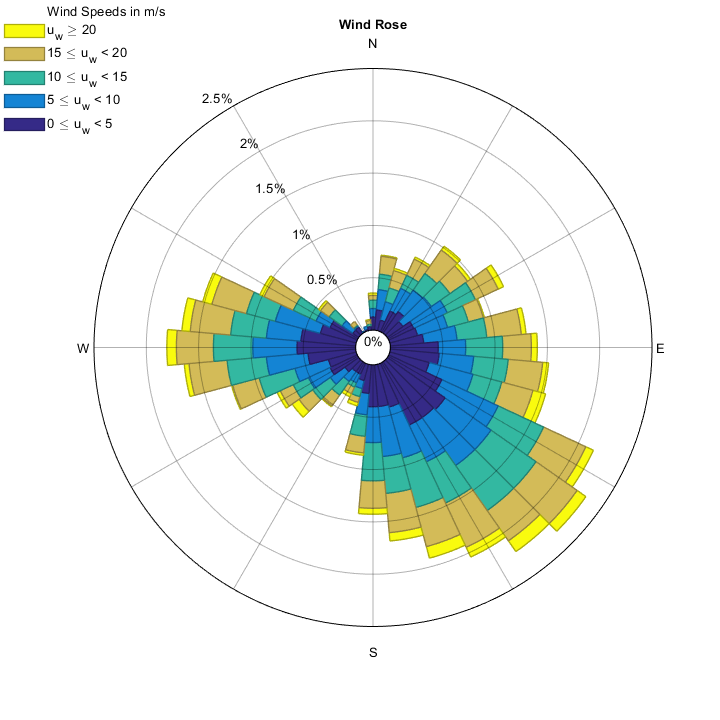The default option represents 36 directions (with an aperture angle of 360/36 = 10º each) Note that the bins are centered in+0° (-5° to+5°) by default.

## Reference angles

### 'AngleNorth' and 'AngleEast'

Tell the function your data's references (origin and orientation).

As there will always be controversy about which should be the reference angles, this function uses by default the trigonometric convention:

Counterclockwise, with 0° angle in the right of the circle (East).

If you want to define your own convention, use sexagesimal degrees to define which angle corresponds to North direction (up) and to East direction (right), so any user can use the desired references. These two values must differ in 90° and both values must be specified.

The meteorological convention, where North is 0° and East is 90° (we can then define origin [N] and orientation [clockwise]), is the most usual:

				[figure_handle, count, speeds, directions, Table] = WindRose(dir, spd, 'AngleNorth', 0, 'AngleEast', 90);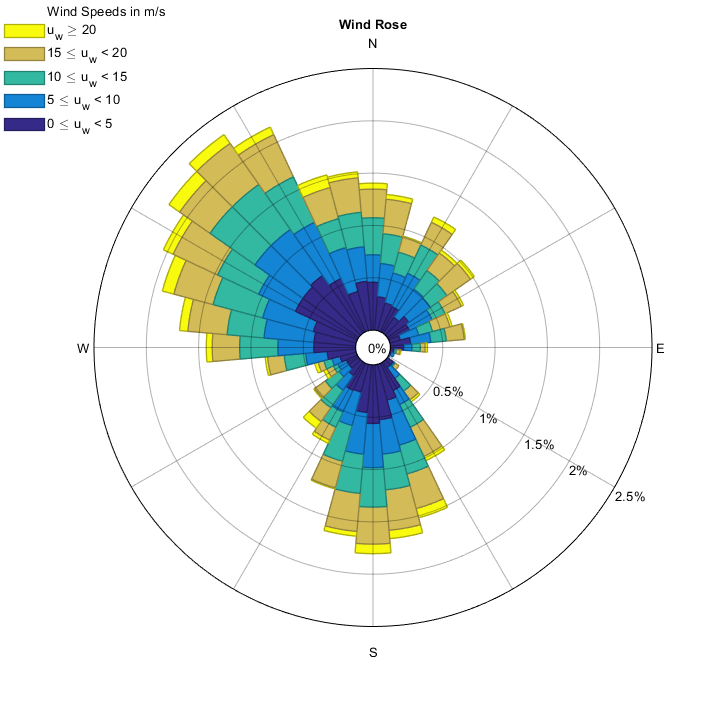Let's imagine that our data uses the noon solar convention in the northern hemisphere (0° is South, and 90° is West)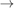North is 180° and East is 270°.

				[figure_handle, count, speeds, directions, Table] = WindRose(dir, spd, 'AngleNorth', 180, 'AngleEast', 270);## Frequency labels

### 'FreqLabelAngle'

Show Frequency label angles.

If we want to know the frequency in each direction, it is recommended to add the frequency labels, in any angle.

This will show the label angle at 60° (measured with the trigonometric reference):

				[figure_handle, count, speeds, directions, Table] = WindRose(dir, spd, 'FreqLabelAngle', 60);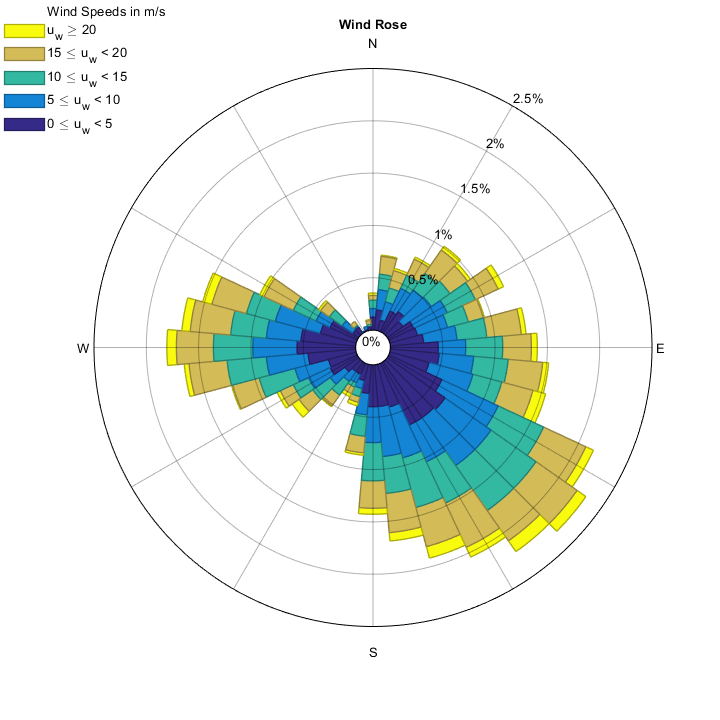Using 'auto' shows the frequency labels on the least frequent direction:

				[figure_handle, count, speeds, directions, Table] = WindRose(dir, spd, 'FreqLabelAngle', 'auto');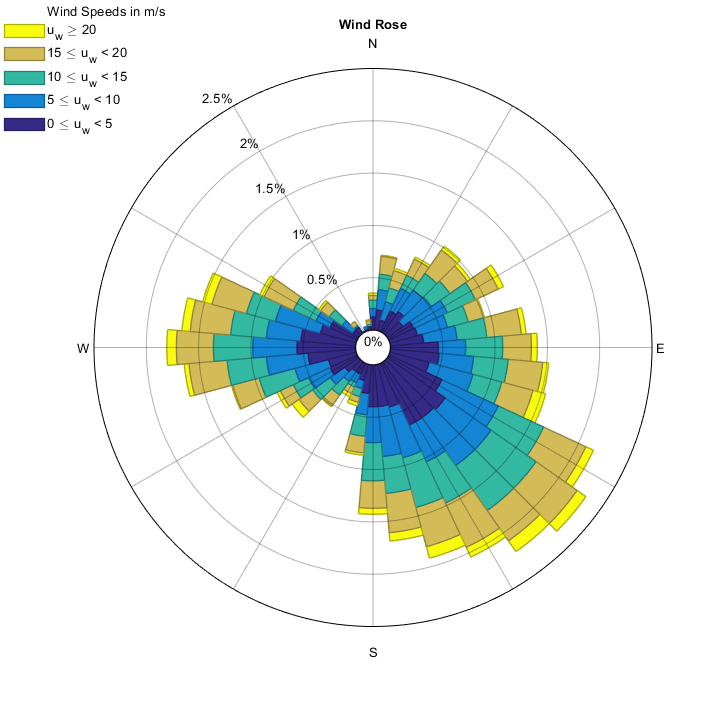Set 'FreqLabelAngle' to 'none', NaN or [] to prevent the frequency labels from appearing.

				[figure_handle, count, speeds, directions, Table] = WindRose(dir, spd, 'FreqLabelAngle', 'none');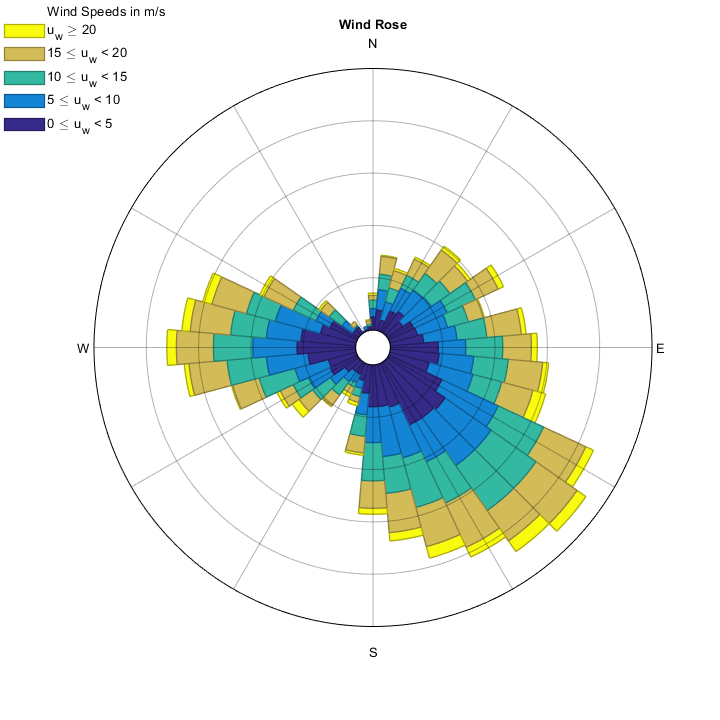Set 'FreqLabelAngle' to 'ruler', 'rulerRight' or 'rulerLeft' to show the frequency bar in a ruler style.

				[figure_handle, count, speeds, directions, Table] = WindRose(dir, spd, 'FreqLabelAngle', 'ruler');[figure_handle, count, speeds, directions, Table] = WindRose(dir, spd, 'FreqLabelAngle', 'rulerLeft');


## Number of directions

### 'nDirections'

Set the number of separate directions bins to be calculated and shown.

The number of directions can be changed by adding the number of directions we want to show, let's say, 8, by specifying 'ndirections'.

				[figure_handle, count, speeds, directions, Table] = WindRose(dir, spd, 'nDirections', 8);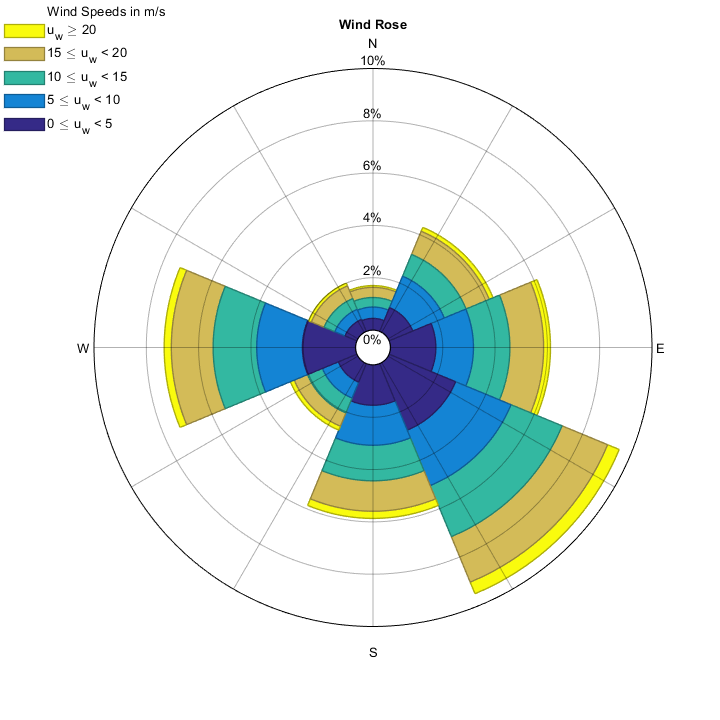We can also specify labels for these directions:

				[figure_handle, count, speeds, directions, Table] = WindRose(dir, spd, 'nDirections', 8, 'labels', {'N', 'NE', 'E', 'SE', 'S', 'SW', 'W', 'NW'});Note that the radial grid values are changed when adding a cell array for the 'labels'. See Axis labels, Example 3 for more information.

## Bin alignment

### 'CenteredIn0'

Center of bin can be the center or the origin of the direction interval.

If you do not want the bins (arms) to be centered in+0° direction, but you want them starting at that point, you can specify that bins should not be centered in 0.

This example is much clearer with a reduced number of directions:

				[figure_handle, count, speeds, directions, Table] = WindRose(dir, spd, 'nDirections', 8, 'CenteredIn0', false);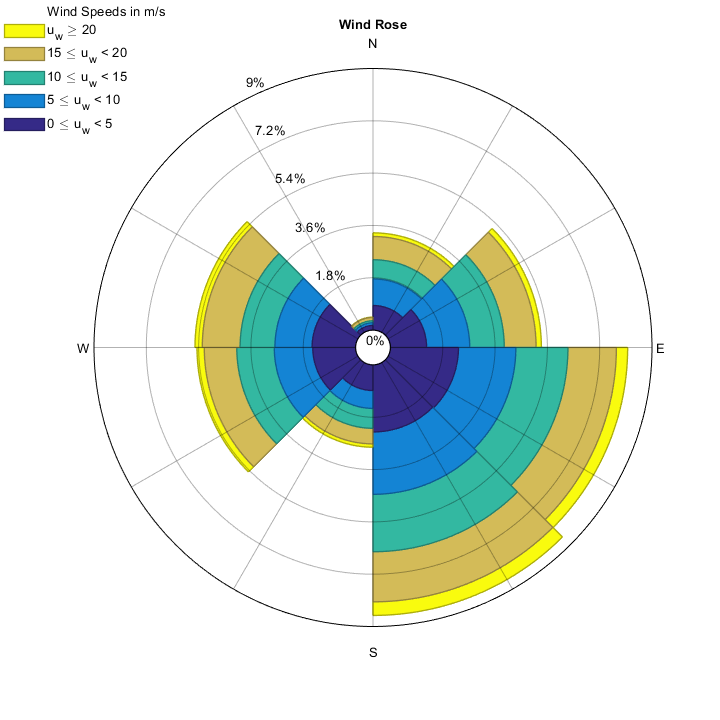Compare the bins to the previous examples. In this new figure, bins appear in the direction 0° to 45° (360°/8), centered in 22.5°. They would normally appear between -22.5 and 22.5, centered in 0 if the parameter 'CenteredIn0' is set to true or omitted (default is true).

The wind rose computes the number of pointing directions in this new range, so the figure is not just rotated with respect to the original: the values are calculated in a different way, because they take into consideration different direction values.

## Speed/intensity ranges number

### 'nSpeeds'

Choose how many speed/intensity intervals should appear. An equispaced interval will be created.

Not only can the direction bins be modified. The number of bins for the intensities can be also changed:

				[figure_handle, count, speeds, directions, Table] = WindRose(dir, spd, 'nSpeeds', 4);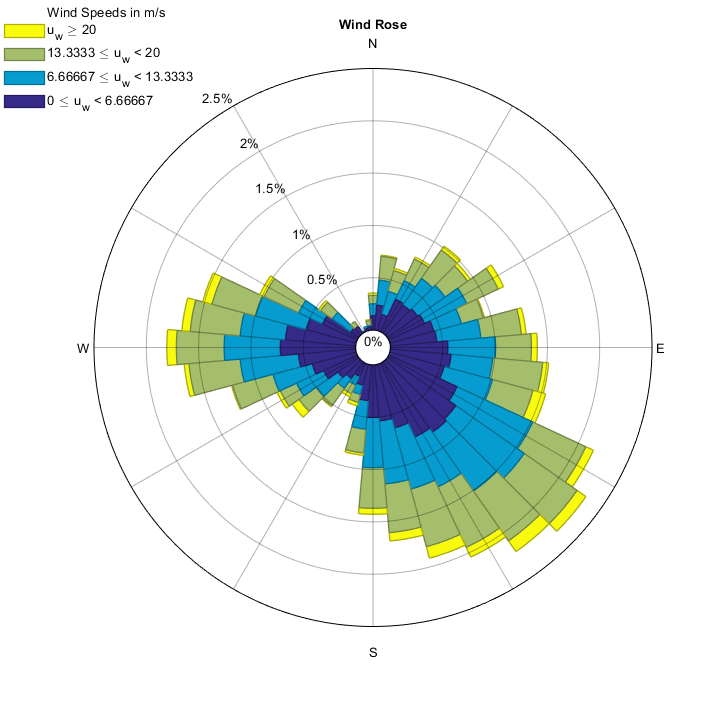It is very common that the maximum intensity bin does not appear in the graph (this depends on the frequency and the rounded value of the maximum).

## Speed/intensity ranges values

### 'vWinds'

Choose the values of the speed/intensity intervals. Allows creating non-equispaced intervals.

If you prefer defining the speed values to create the bins, you can also do that with 'vWinds'(this will omit the 'nspeeds' command, so use whichever you need). Beware of this, since you have to know the speed ranges, in order to prevent possible errors or bins not appearing.

				[figure_handle, count, speeds, directions, Table] = WindRose(dir, spd, 'vWinds', [0 0.5 1 1.2 2 5]);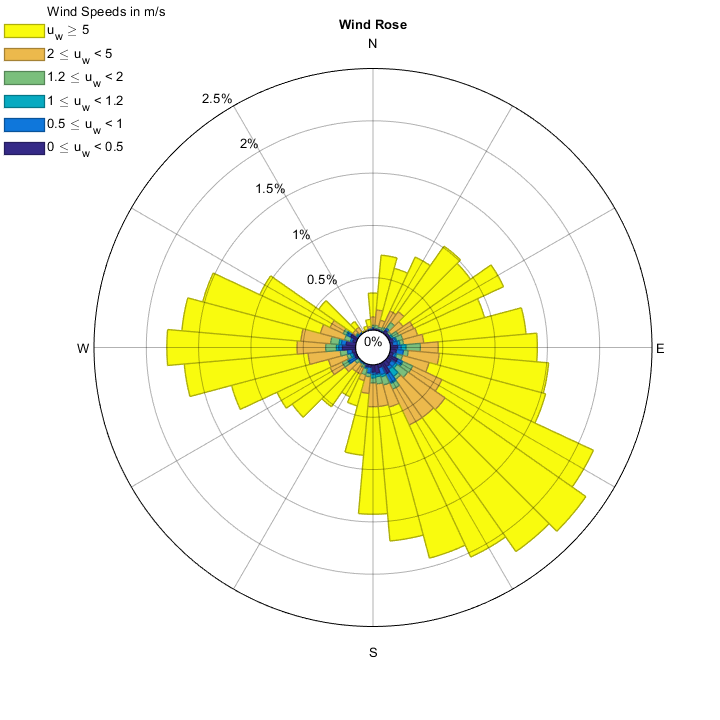## Round maximum speed/intensity

### 'SpeedRound'

Round the maximum speed value to a specific number.

If the maximum intensity is not defined as you wanted, change this (let's imagine that you wanted the maximum speed to be multiple of 40, with 6 intensity ranges, so all the speed ranges have integer values):

				[figure_handle, count, speeds, directions, Table] = WindRose(dir, spd, 'SpeedRound', 40, 'nSpeeds', 6);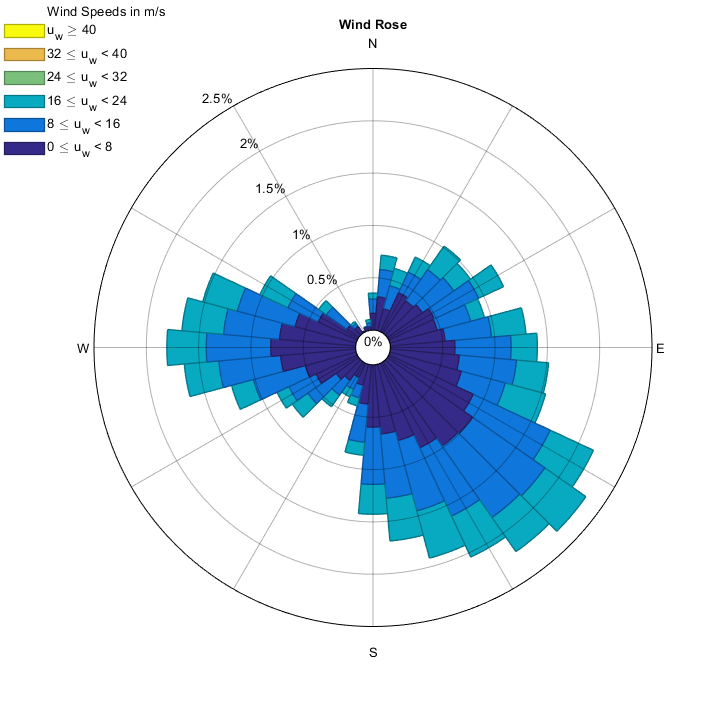## Maximum frequency

### 'MaxFrequency'

Define the limit of the plot, extending the maximum frequency to this value.

The bins are drawn in a way that they fit inside the maximum circle, but, in order to compare different wind roses, it could be interesting to keep a fixed value for the maximum frequency (in percentage, 0-100).

Let's add the frequency labels too, selecting the position automatically, so we can see the frequencies that are calculated in this case and that the maximum frequency is set to 6%.

				[figure_handle, count, speeds, directions, Table] = WindRose(dir, spd, 'MaxFrequency', 6, 'FreqLabelAngle', 'auto');## Frequency/circular grid lines

### 'nFreq'

Display the specified number of frequency/circular grid lines.

In order to check the frequencies in the final wind rose, it can be hard if data are very scattered. We can add as many frequency circles as we want. This example adds 10 frequency grid lines and the labels in the 90° (trigonometric) line.

				[figure_handle, count, speeds, directions, Table] = WindRose(dir, spd, 'nFreq', 10, 'FreqLabelAngle', 90);## Frequency/circular grid lines values.

### 'freqs'

Display the frequency/circular grid lines at specific values.

			    [figure_handle, count, speeds, directions, Table, Others] = WindRose(dir, spd, 'freqs', [0.4 1 2 2.5], 'FreqLabelAngle', 'auto');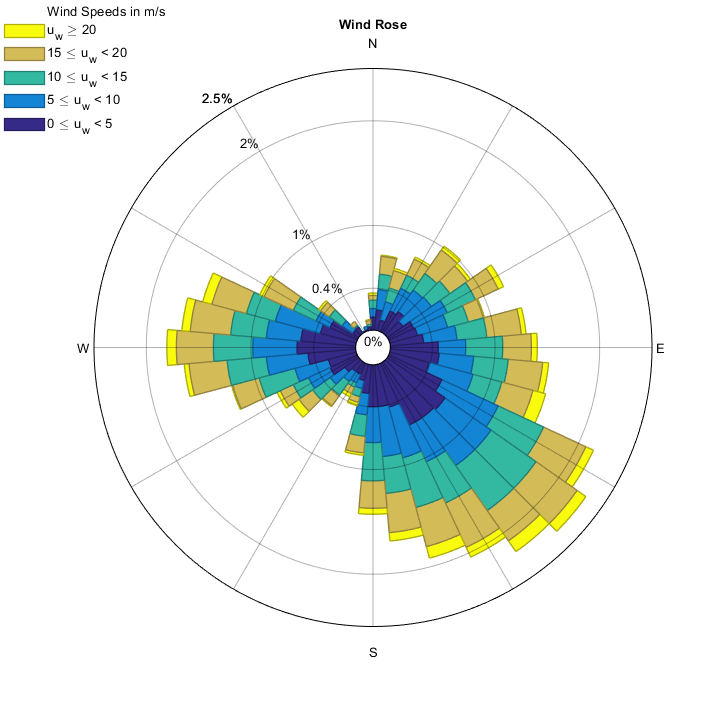## Radial grid number of divisions

Display this number of radial/sector divisions.

				[figure_handle, count, speeds, directions, Table] = WindRose(dir, spd, 'radialgridnumber', 24);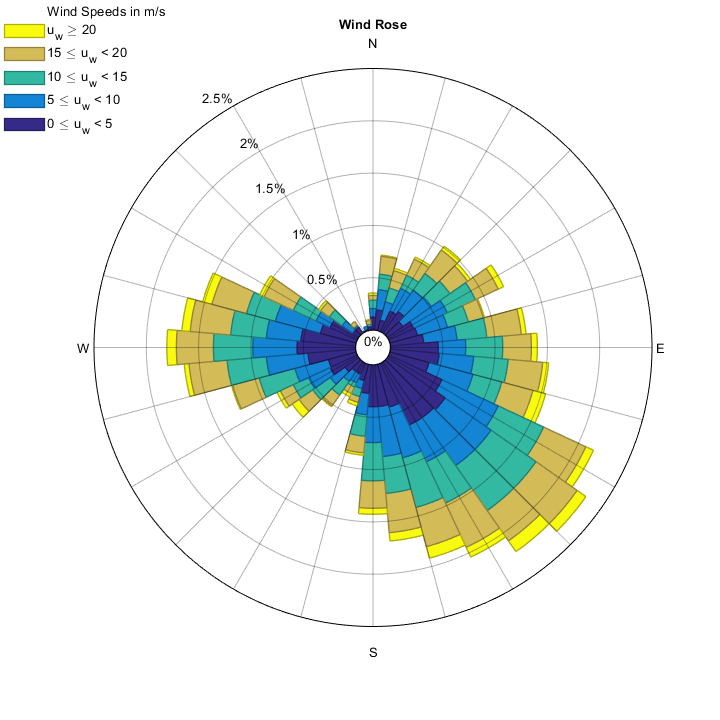## Round maximum frequency value

### 'FreqRound'

Round the maximum frequency to the input value.

The maximum frequency value can be rounded to a desired figure, in order to compare very different wind roses, while keeping a uniform style.

Frequency labels are shown, in order to better understand this effect.

In this example, the maximum frequency will be the lowest integer multiple of 2 which allows showing all the data:

[
figure_handle, count, speeds, directions, Table] = WindRose(dir, spd, 'FreqRound', 2, 'FreqLabelAngle', 45);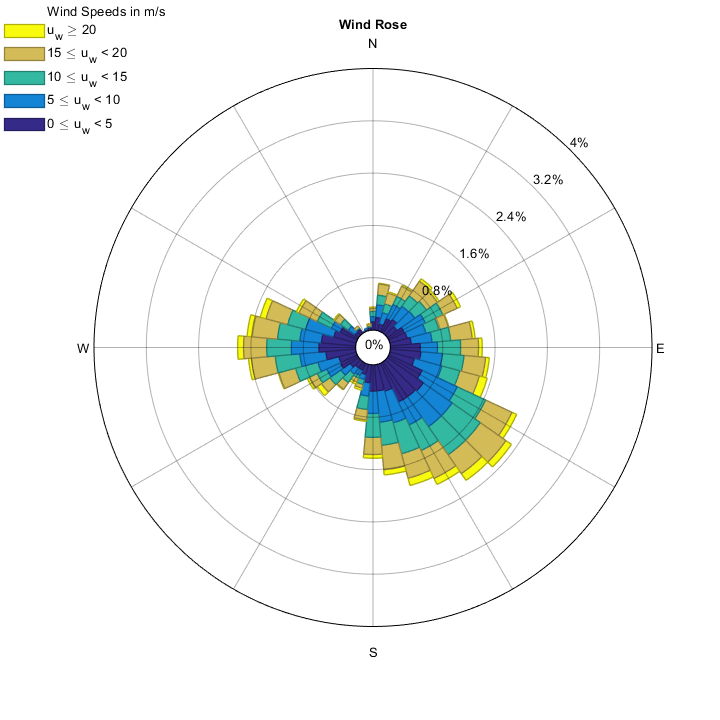## Lowest speed bin outside

### 'inverse'

Display the lowest speed bins outside the wind rose, and the highest speeds inside.

If you want to show the lowest speeds at the outermost part of the wind rose, there is a possibility to do this, using 'inverse', true

				[figure_handle, count, speeds, directions, Table] = WindRose(dir, spd, 'inverse', true);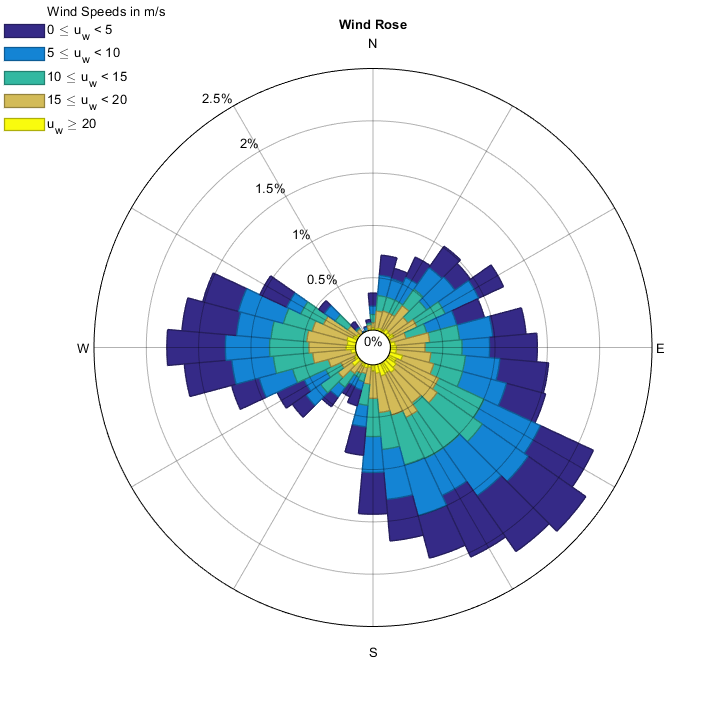## Title, legend label and legend magnitude

### 'TitleString', 'LabLegend', 'LegendVariable'

Change the default text strings in the plot, other than labels.

If you are using WindRose for other representation that does nothing to do with wind, you can change the title, the legend's label and the variable. You can use TeX strings too.

This example could be valid for a router or for a directional microphone:

				d_r = linspace(0,350,36); int = 3*sind(-d_r)+3.5; int = int/max(int);
DIR = []; INT = []; for i=1:length(d_r); DIR = [DIR;repmat(d_r(i),round(100*int(i)),1)]; INT = [INT;repmat(100*int(i)-130,round(100*int(i)),1)]; end

[figure_handle, count, speeds, directions, Table] = WindRose(DIR, INT, 'TitleString', {'COMTREND R252a';''}, 'LabLegend', 'Signal strength in dBm', 'LegendVariable', 'I_0' ,'zeroAndNegative', true);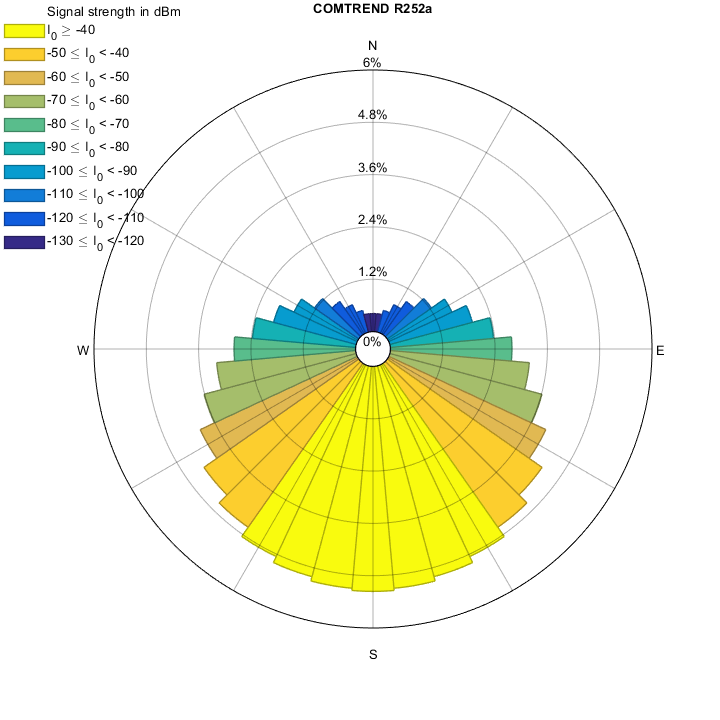## Negative and zero values consideration

### 'zeroAndNegative'

Consider negative and zero values.

Wind does not present negative values, and zeros shall be omitted from the drawing. If this is not your case, and you want negative and zero values to appear in the wind rose, set 'zeroAndNegative',false. Default is true, so you don't have to worry about this.

				[figure_handle, count, speeds, directions, Table] = WindRose(DIR, INT, 'zeroAndNegative', true);


Set the minimum relative radius of the plot.

Some users do not like wind roses with a hole in the middle, but others like it a bit wider. Change this attribute by changing the min_radius value, which is relative to the circle radius.

Radius In this function is defined as: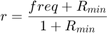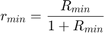Where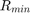is the 'min_radius' value and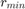is the actual minimum radius plotted, relative to the maximum wind rose radius.

In order to get a hole 25% of the total, use 0.25/(1-0.25) = 1/3 as min radius.

The default value for min_radius is 1/15, which implies a hole (1/15)/(1+1/15) = 1/16 of the circle).

This example uses a minimum radius of 0 (no hole at the center):

				[figure_handle, count, speeds, directions, Table] = WindRose(dir, spd, 'Min_Radius', 0, 'TitleString', 'Min Radius = 0');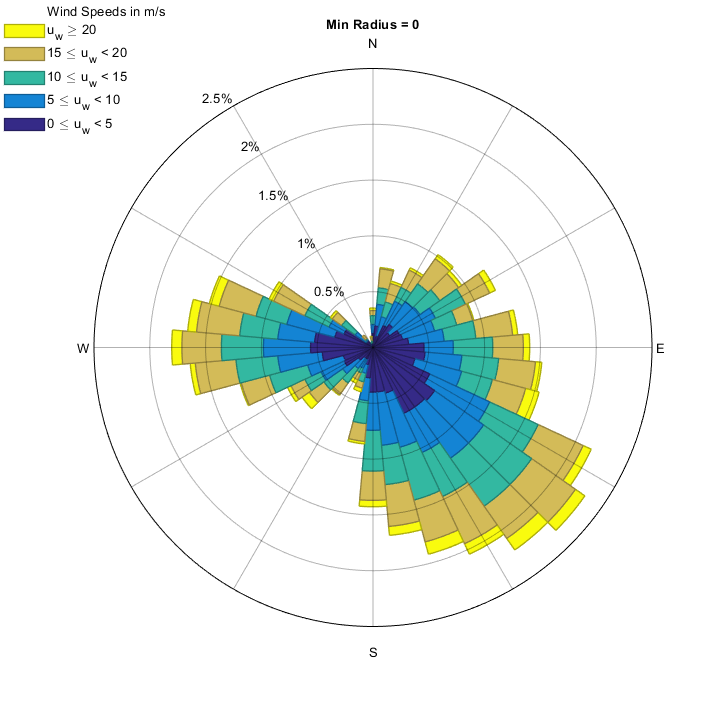This example uses a minimum radius of 0.5 (the inner hole is 0.5/(1+0.5) = 1/3 of the radius of the wind rose):

				[figure_handle, count, speeds, directions, Table] = WindRose(dir, spd, 'min_radius', 0.5, 'TitleString', 'Min Radius = 0.5');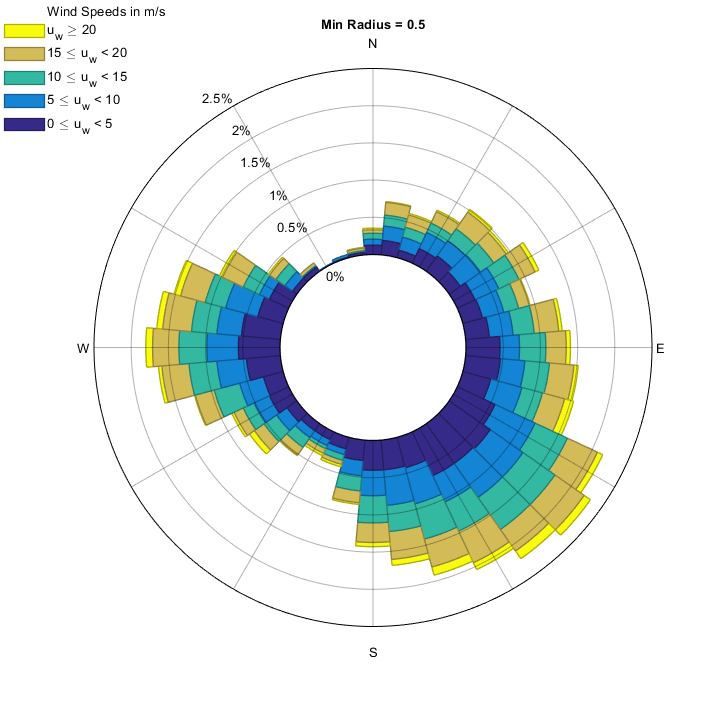This example uses a minimum radius of 9 (the inner hole takes 9/(1+9) = 90% of the wind rose):

				[figure_handle, count, speeds, directions, Table] = WindRose(dir, spd, 'min_radius', 9, 'TitleString', 'Min Radius = 9');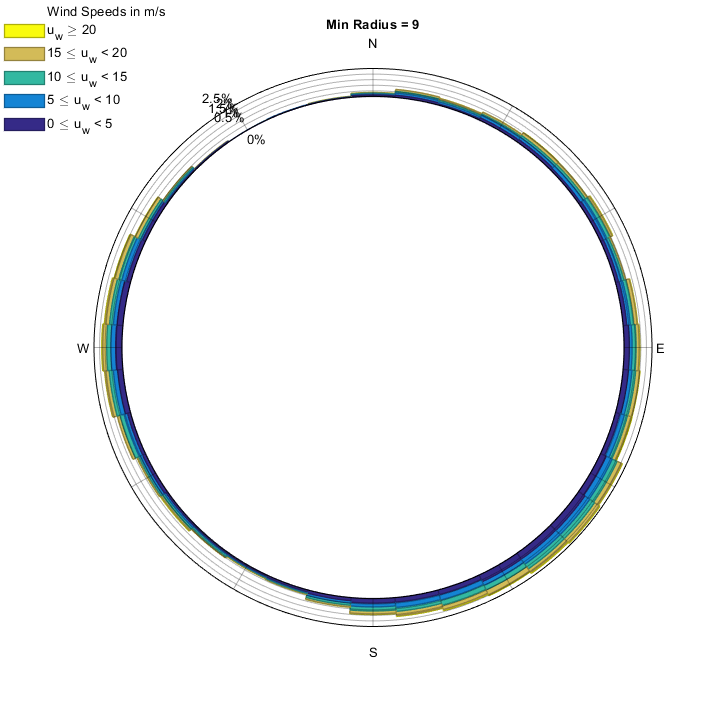## Colormap

### 'cMap'

Change the color scheme for this wind rose.

If you hate the "parula or jet" color maps -default, depending on whether you have parula available or not-, you can use any other built in color map you want, even if it is defined in a Matlab function in your working paths (this means that the function you use does not necessarily be part of Matlab) You can also invert the color map just by adding "inv_" at the beginning of the color map name.

In this example, we will use the "copper" color map but inverted:

				[figure_handle, count, speeds, directions, Table] = WindRose(dir, spd, 'cMap', 'inv_copper');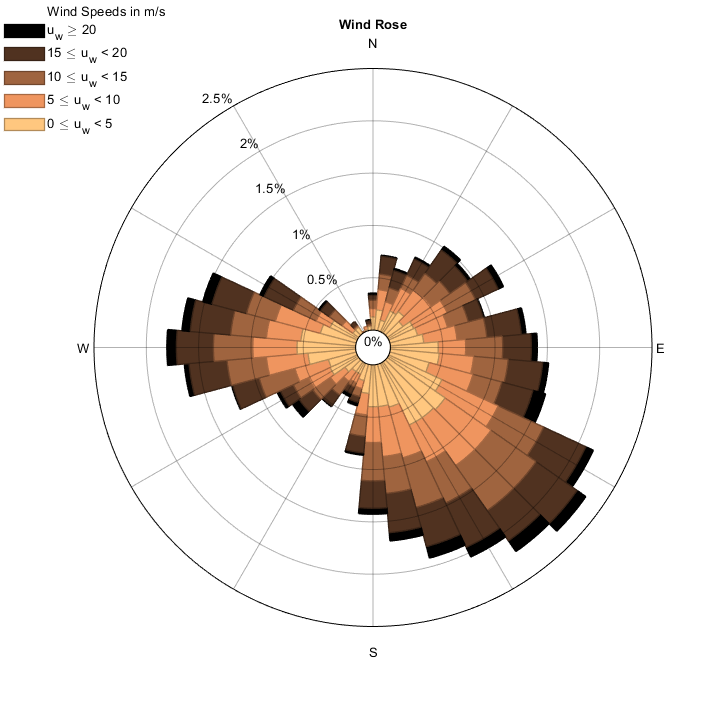But we can also use my favorite color map, "magma", which I have as a file in my Matlab path:

				[figure_handle, count, speeds, directions, Table] = WindRose(dir, spd, 'cMap', 'magma');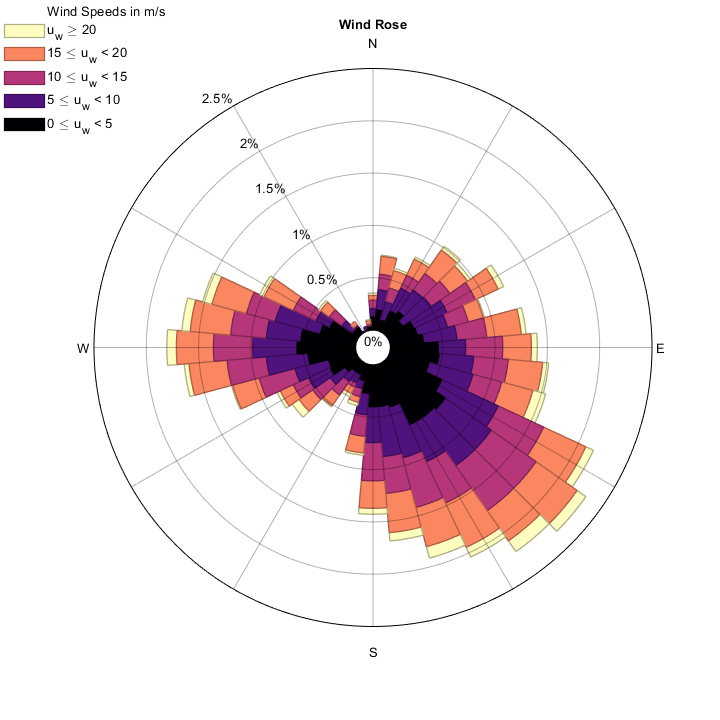We can also invert this color map by calling it invmagma (despite invmagma is not an actual function):

				[figure_handle, count, speeds, directions, Table] = WindRose(dir, spd, 'cMap', 'inv_magma');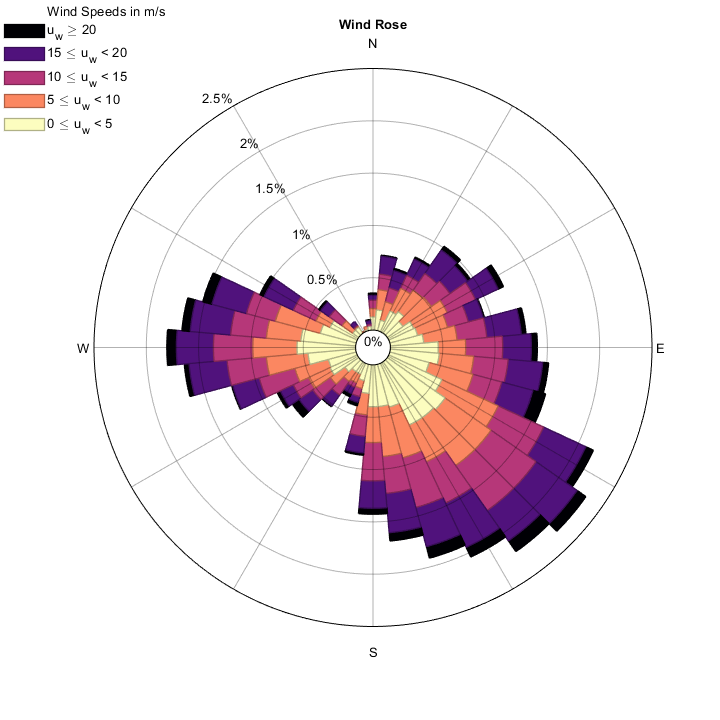You can also use a color map of your own by using a n×3 array. This, compared to the following option, does not need setting the number of speeds, but may result in something different from what you expect, since these are inteporlated from the data you pass to the function.

				mycolormap = [0 0 1; 0 1 1; 0 1 0; 1 1 0; 1 0 0]; % Blue, Cyan, Green, Yellow, Red
[figure_handle, count, speeds, directions, Table] = WindRose(dir, spd, 'cMap', mycolormap);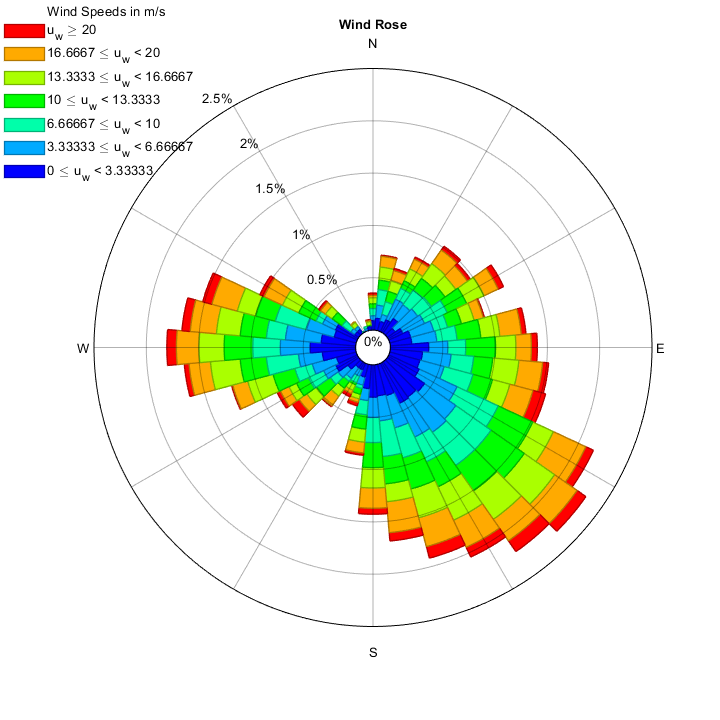Note that despite we put yellow in the color map, it does not appear finally, since the final colormap is an interpolation of 7 colors from the 5 we specified. See the next section for a different approach.

## Colors

### 'colors' + 'nSpeeds' or 'vWinds'

Set specific colors for specific intervals.

If you specify the 'nSpeeds' or the 'vWinds' argument to define the intensity intervals, a customized and fixed final colormap without interpolation can be used, specifying a numeric array for 'colors'.

It is compulsory to specify the number of speed bins, and must match the number of colors.

If we want the 5 colors we specified in the previous section to appear without interpolation, this is the appropriate function call:

				mycolormap = [0 0 1; 0 1 1; 0 1 0; 1 1 0; 1 0 0]; % Blue, Cyan, Green, Yellow, Red
[figure_handle, count, speeds, directions, Table] = WindRose(dir, spd, 'nSpeeds', 5, 'colors', mycolormap);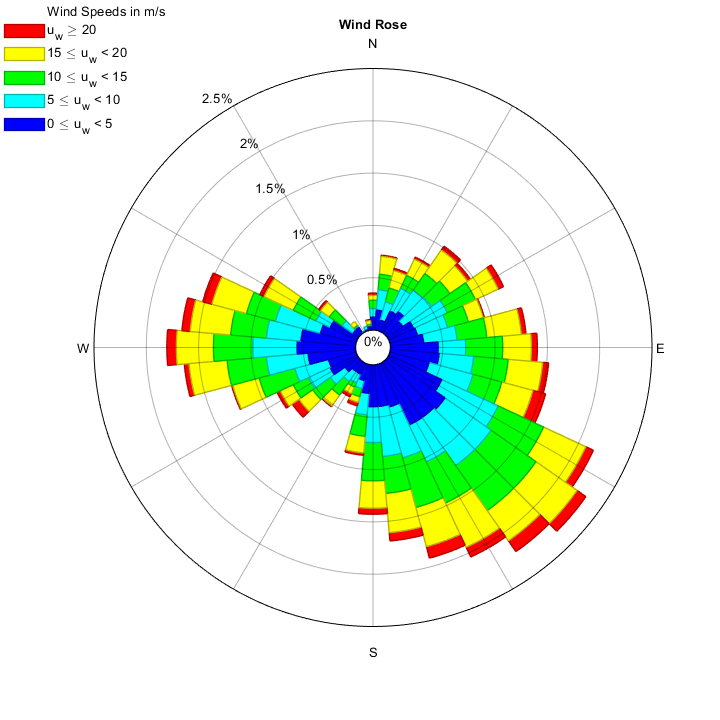In fact, it is the same as using 'cmap' with 'nspeeds' instead of 'colors' with 'nspeeds':

				mycolormap = [0 0 1; 0 1 1; 0 1 0; 1 1 0; 1 0 0]; % Blue, Cyan, Green, Yellow, Red
[figure_handle, count, speeds, directions, Table] = WindRose(dir, spd, 'nSpeeds', 5, 'cMap', mycolormap);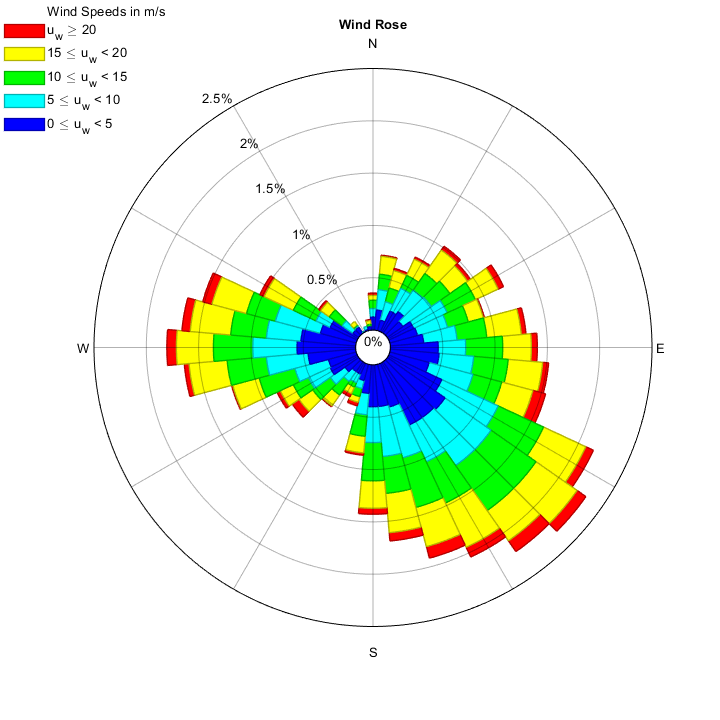This method is more interesting than using a colormap if you want to remark certain speed range. Using "legendtype",1 does not make much sense for custom colors, but it does in custom colormap (previous section). See "Changing the colormap" in previous section for more coloring options:

				mycolormap      = bone(5);
mycolormap(3,:) = [1 0 0];
[figure_handle, count, speeds, directions, Table] = WindRose(dir, spd, 'nSpeeds', 5,'colors', mycolormap);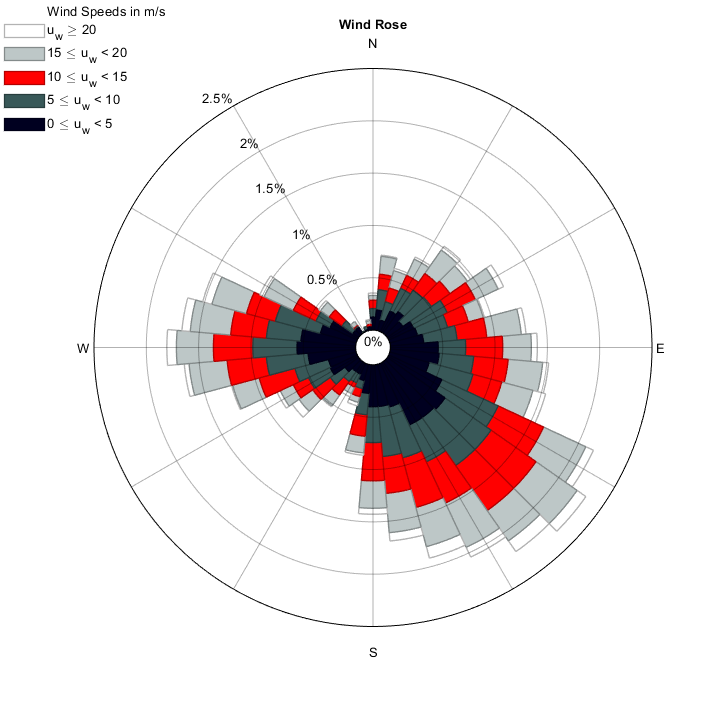## Legend

### 'LegendType'

Change the legend type or remove the legend.

The legend can be two types: Boxes legend -default- ('LegendType',2) or color bar legend ('LegendType',1). From version 2021.04.20, the variable name also appears in colorbar legend.

If you do not want the legend to appear, consider there is an extra type ('LegendType',0):

				[figure_handle, count, speeds, directions, Table] = WindRose(dir, spd, 'LegendType', 1);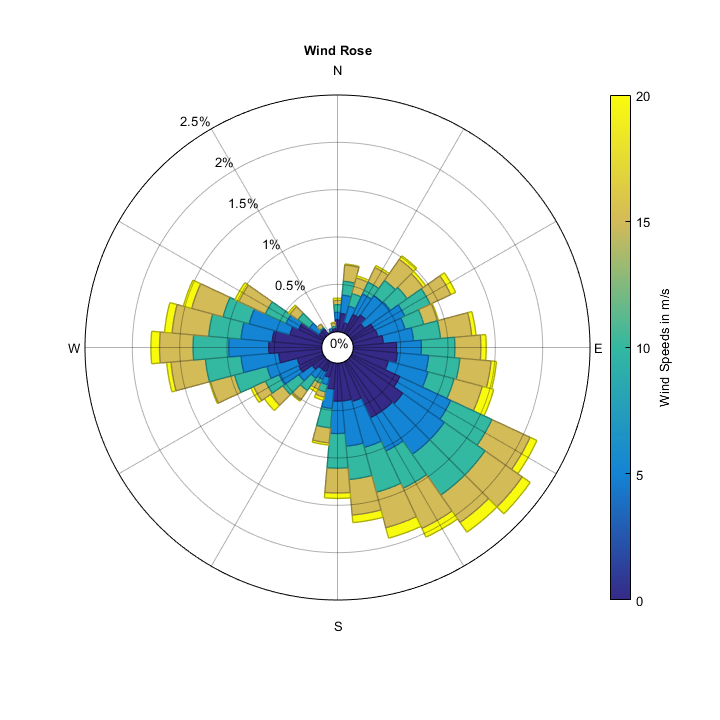## Legend position and orientation

### 'LegendPosition', 'LegendOrientation'

Change the legend's position and orientation.

The box type legend ('LegendType',2) can be positioned anywhere ('north','northeast','east','southeast','south','southwest','west','northwest').

The colorbar type legend ('LegendType',1) can be positioned in 'north','east','south','west' The default position for box type is 'northwest' and the default position for colorbar is 'east'.

For the box type legend, the orientation can also be changed (horizontal or vertical).

For the colorbar type, the orientation is automatically defined: horizontal for north and south positions, vertical otherwise.

			    [figure_handle, count, speeds, directions, Table, Others] = WindRose(dir, spd, 'LegendType', 2, 'LegendPosition', 'south', 'LegendOrientation', 'vertical');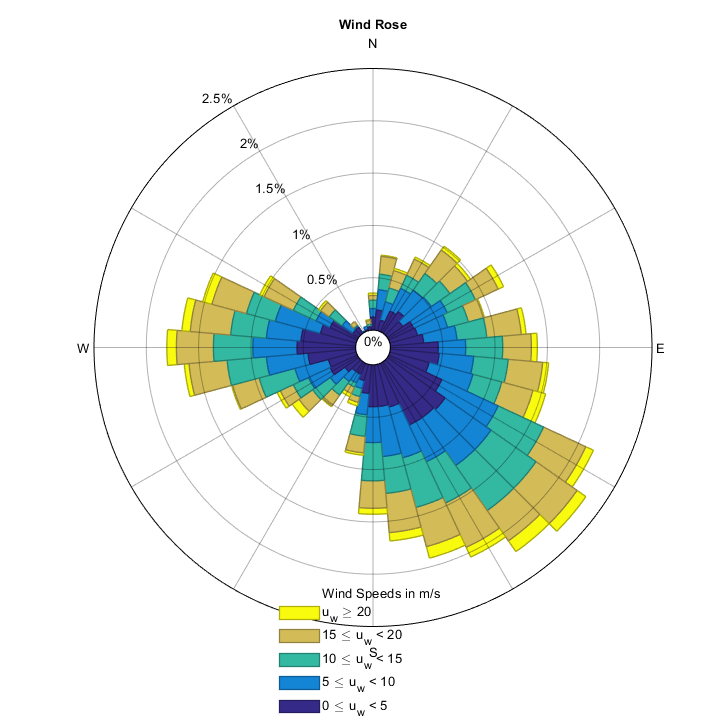[figure_handle, count, speeds, directions, Table, Others] = WindRose(dir, spd, 'LegendType', 2, 'LegendPosition', 'southeast', 'LegendOrientation', 'horizontal');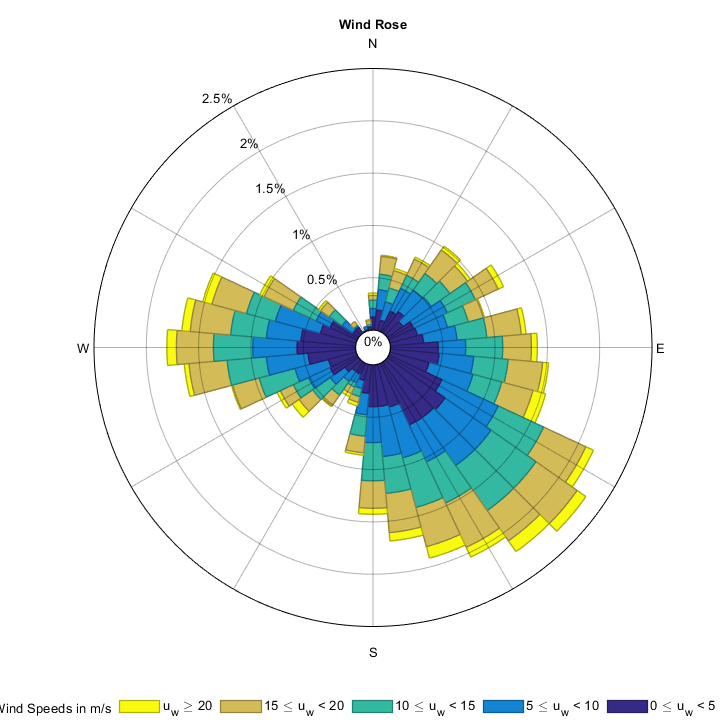[figure_handle, count, speeds, directions, Table, Others] = WindRose(dir, spd, 'LegendType', 1, 'LegendPosition', 'west');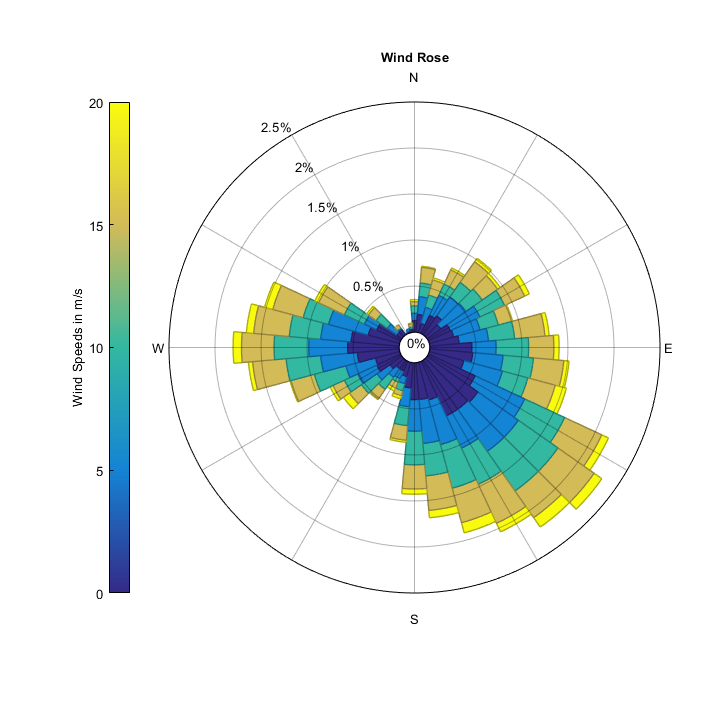[figure_handle, count, speeds, directions, Table, Others] = WindRose(dir, spd, 'LegendType', 1, 'LegendPosition', 'north');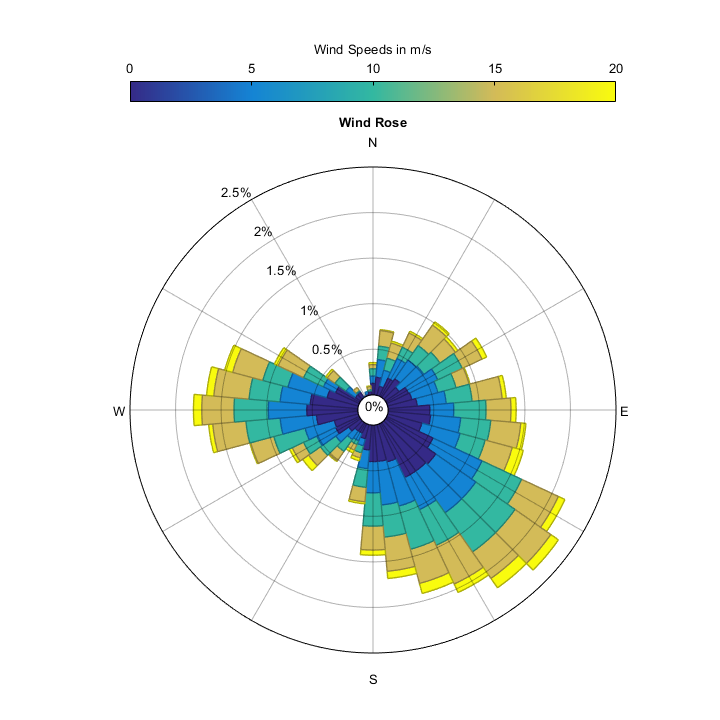## Figure size

### 'width' and 'height'

Change figure inner dimensions.

The default figure will be squared, with the length of the square being 2/3 of the smallest dimension of the screen (usually the height). You can specify the figure dimensions in pixels. These dimensions will be the inner figure dimensions (window border is added internally, so the axes are exactly the size you define):

				[figure_handle, count, speeds, directions, Table] = WindRose(dir, spd, 'height', 256, 'width', 512);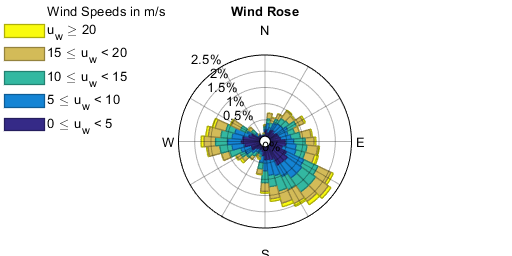## Scale factor

### 'scalefactor'

Change the size of the wind rose inside the figure.

If you consider that the wind rose is too big inside the figure, you can reduce its size by using an scale factor (0 to 1):

				[figure_handle, count, speeds, directions, Table] = WindRose(dir, spd, 'scalefactor', 0.5);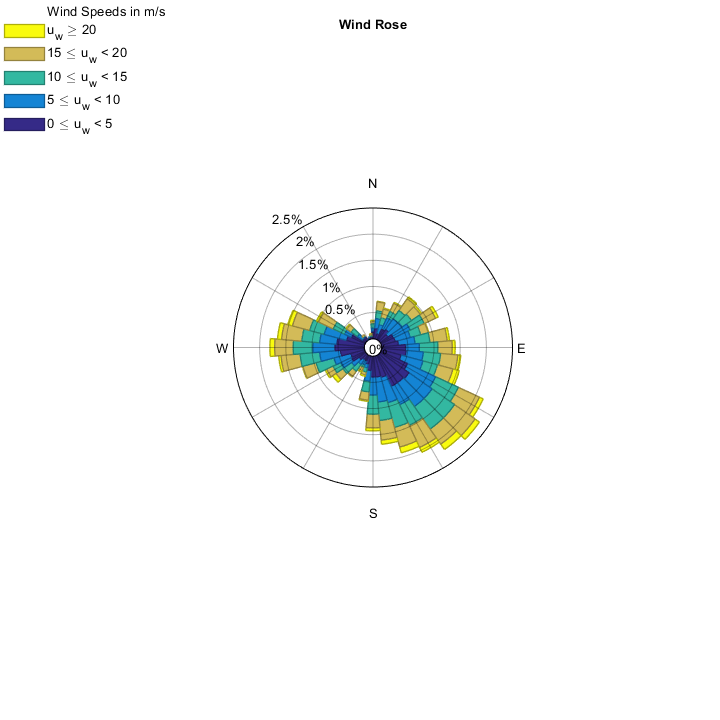## Figure toolbars

Change or hide the figure's menubar and toolbar.

If you want to remove the figure and menu bars, you can use the same instructions used in a normal figure:

				[figure_handle, count, speeds, directions, Table] = WindRose(dir, spd, 'menubar', 'none', 'toolbar', 'none');


## Figure, text and grid colors

### 'figColor', 'textColor', 'gridColor'

Change figure, text or grid colors.

Figure is white by default; while text appears to be black. Modify them as wanted. Use Matlab built-in colors (red 'r', green 'g', blue 'b', white 'w', black 'k', yellow 'y', magenta 'm', cyan 'c') or use custom RGB vector, both for figure, text and grid lines:

				[figure_handle, count, speeds, directions, Table] = WindRose(dir, spd, 'figColor', 'k', 'textColor', [1 1 0.5], 'gridColor', 'w');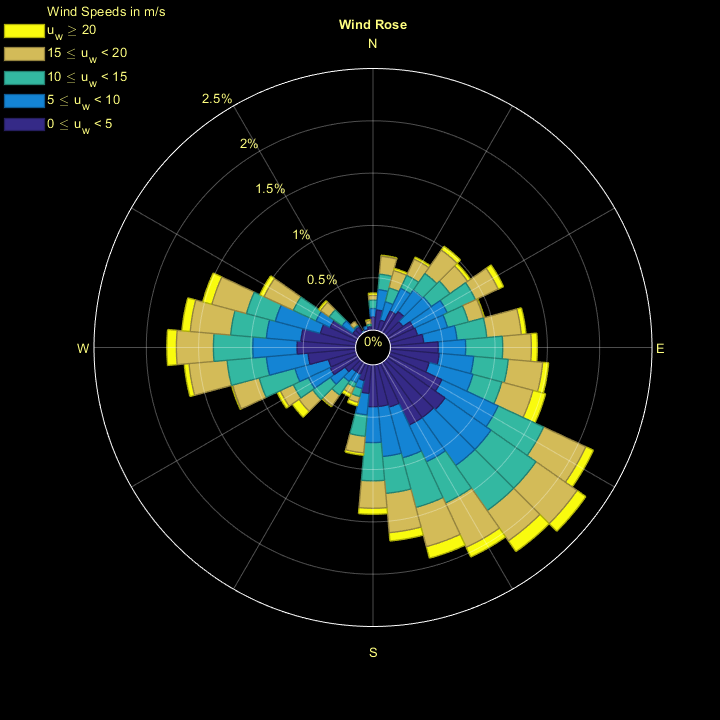## Axis labels

### 'labels'

Change direction labels. Any number of labels is allowed now.

Axis labels - default are N, E, S and W - can be modified with separate specific properties or with a cell array {'Label for North', 'Label for East', 'Label for South', 'Label for West'}

Put labels separately:

				[figure_handle, count, speeds, directions, Table] = WindRose(dir, spd, 'labelNorth', 'North',...
'labelSouth', 'South', 'labelEast', 'East', 'labelWest', 'West');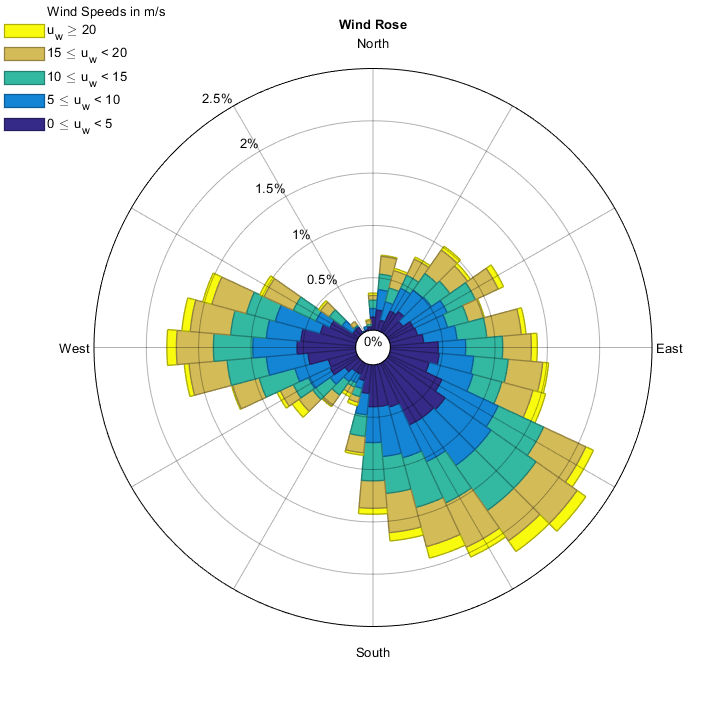Specify labels together (North, East, South, West)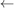NOTE THAT THE ORDER IN 2015 VERSIONS WAS (North, South, East and West):

				[figure_handle, count, speeds, directions, Table] = WindRose(dir, spd, 'labels', {'North (90°)', 'East (0°)', 'South (270°)', 'West (180°)'});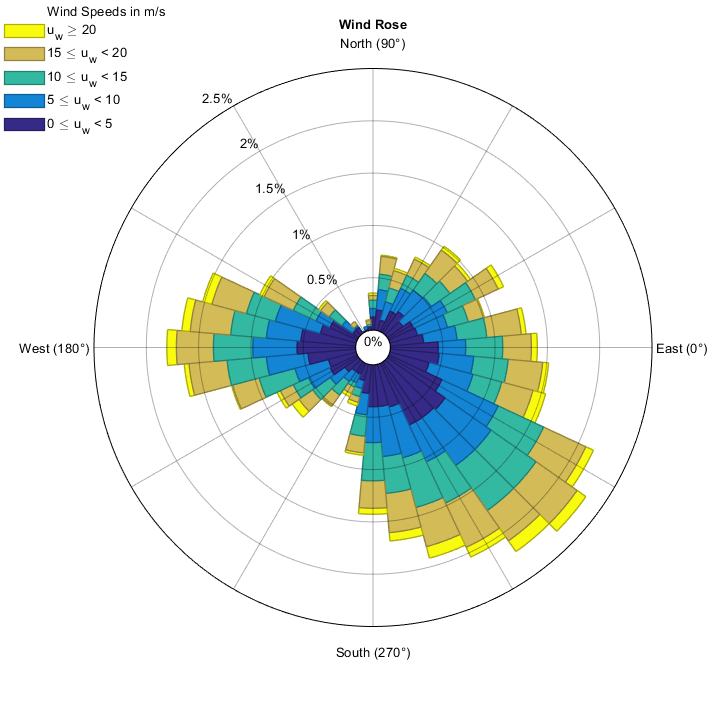If we specify any other number of labels in the form of a cell array, these will be distributed from the top, clockwise. Note that the radial divisions are automatically set to the number of labels (unless 'radialgridnumber' is set to a different value). Any string (empty characters too) can be put into this cell array, thus omitting that label in the graph. See the example below:

				[figure_handle, count, speeds, directions, Table] = WindRose(dir, spd, 'labels', {'N', '', 'This is North-East', 'ENE',...
'E', 'ESE', 'SE - Note!', 'SSE', 'S', 'SSW', 'This is not North-East','WSW', 'W', '', 'NW', 'NNW'});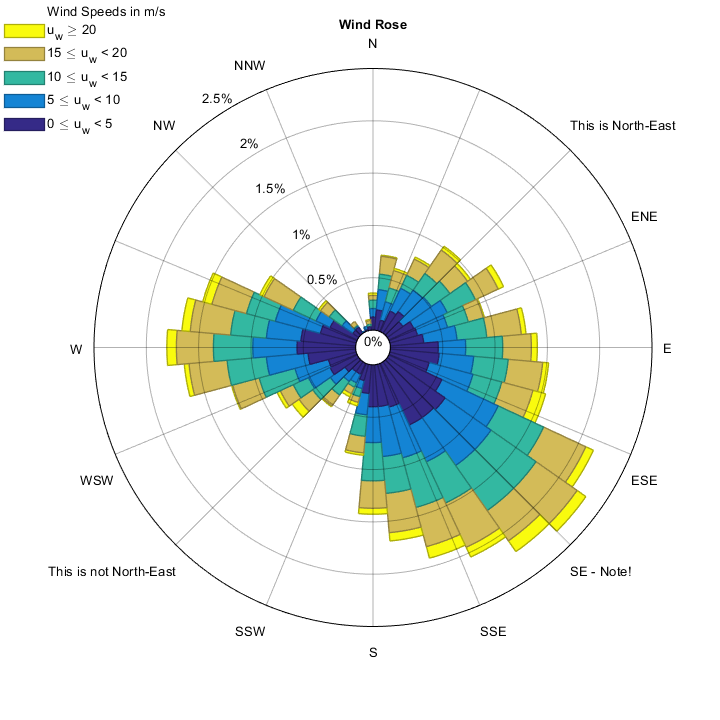Note that 16 grid lines are automatically added for the 16 labels specified.

## Grid line styles

### 'gridStyle', 'gridColor', 'gridWidth', 'gridalpha'

Change the grid line style, color, width and opacity.

Grid lines can be styled together or separately, indicating line color ('gridColor') , line style ('gridStyle'), line width ('gridWidth') and line alpha (opacity) ('gridalpha') (alpha is only available for Matlab versions 8.4 or greater).

Style grid lines (radial and circular) together:

				Options1 = {'gridstyle', ':', 'gridcolor', 'b', 'gridwidth', 2, 'gridalpha', 0.5};
[figure_handle, count, speeds, directions, Table] = WindRose(dir, spd, Options1);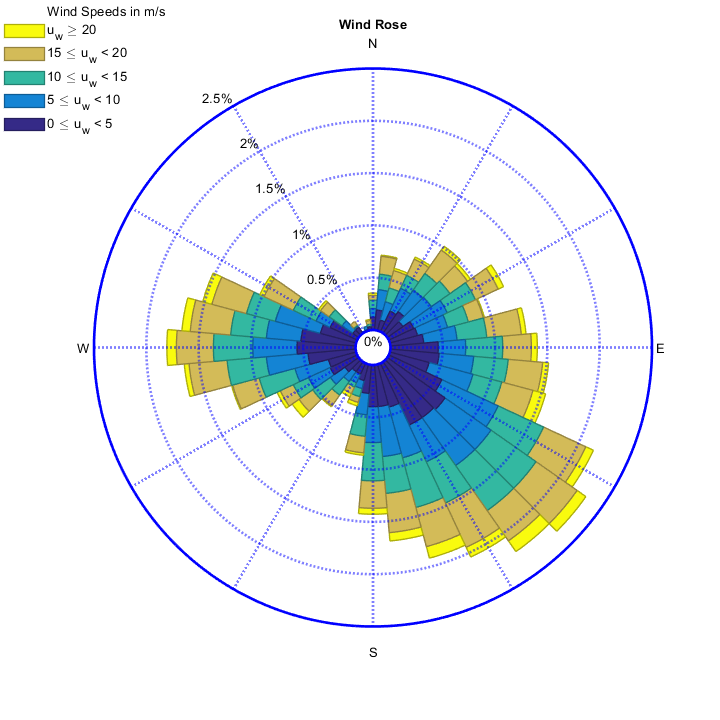Or style them separately:

				Options2 = {'radialgridstyle', '-.', 'circulargridstyle', '--', ...
'radialgridcolor', 'r', 'circulargridcolor', [0 0.7 0]...
, 'radialgridwidth', 1, 'circulargridwidth', 2, ...
[figure_handle, count, speeds, directions, Table] = WindRose(dir, spd, Options2);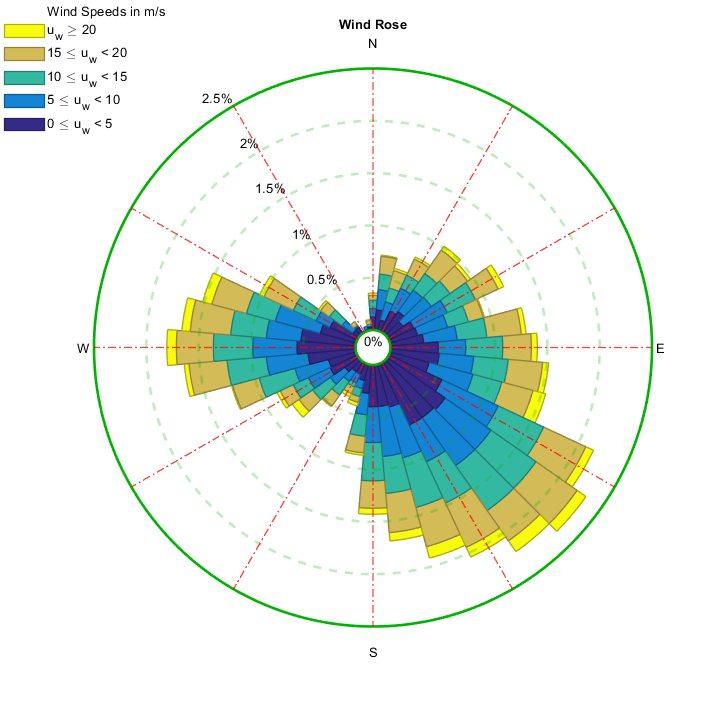## Normalize

### 'normalize'

Normalize frequencies for each direction => Make all arms to extend to the outer ring, Displaying the frequency of each arm

			    [figure_handle, count, speeds, directions, Table, Others] = WindRose(dir, spd, 'normalize', true,'labels','');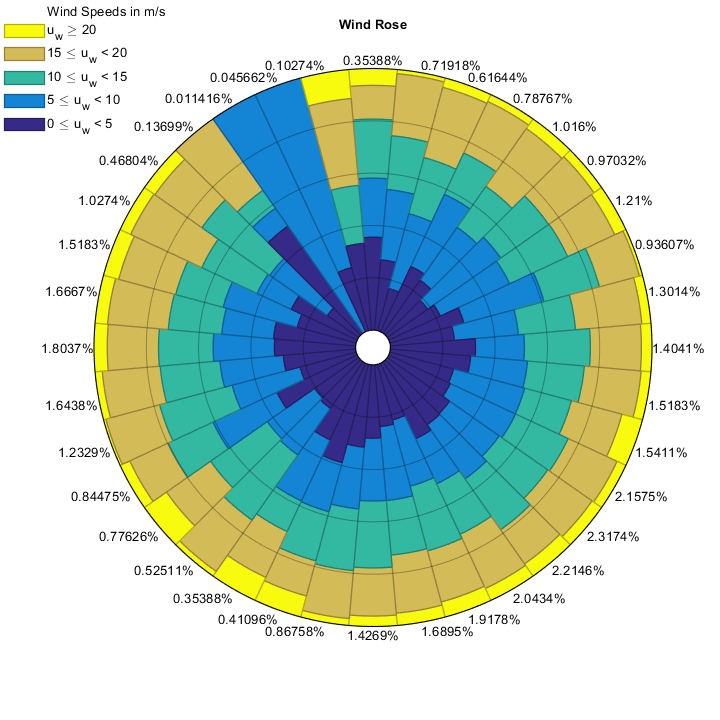## Logarithmic scale for frequencies

### 'logscale'

Display the frequencies grid spacing using a logarithmic function

If some directions are much more frequent than others, maybe a logarithmic scale for the frequency is desirable. The non-linearity of the grid can be changed using the 'logfactor' parameter (see next paragraph).

			    x = linspace(0,360,37);
y = 87*(x==20) + 10*(x==140) + 1*(x==270) + 1*(x==180) + 1*(x==300);
DIR = [];
SPD = [];
for i=1:length(x)
n   = round(y(i)*100); % abs(round(y(i)*100+(2*rand-1)*10));
DIR = [DIR;repmat(x(i),n,1)];<
SPD = [SPD;abs(rand(n,1)*10)];
end
[figure_handle, count, speeds, directions, Table, Others] = WindRose(DIR, SPD, 'logscale', false);
% Note that the logscale option may add some frequency circles compared to the default behaviour. This does not occur when inputting the frequency values or the number of frequencies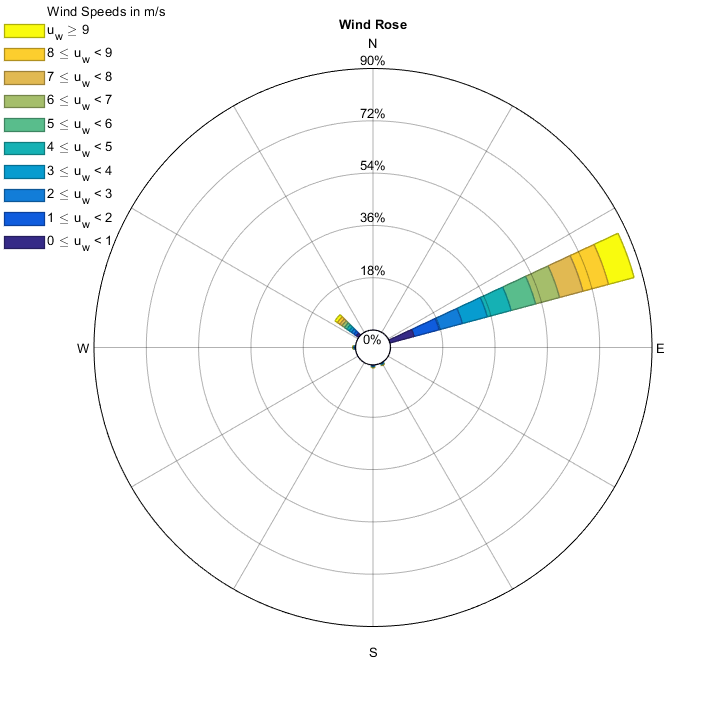[figure_handle, count, speeds, directions, Table, Others] = WindRose(DIR, SPD, 'logscale', true);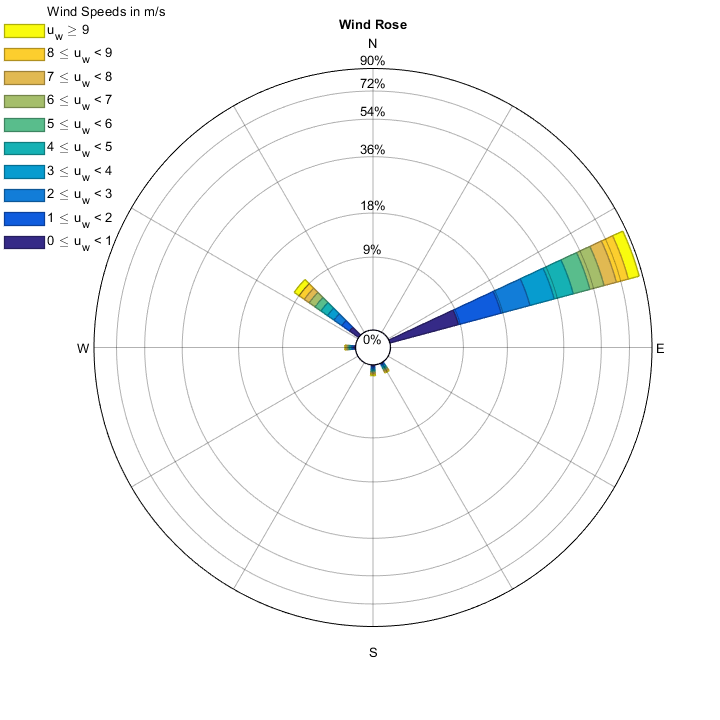## Logarithmic scale parameterized for frequencies

### 'logfactor'

Increase or decrease the non-linearity of the log scale.

The default 'logfactor' for the log scale of frequencies is 10. If you want to increase the non-linearity of the grid, use a higher 'logfactor', for example 100 or 1000. Higher values of 'logfactor' will squeeze high frequencies, and lower values will resemble more and more to linear behaviour. 'logfactor' must be greater than 1, because a logfactor=1 is exactly the same as linear scale ('logscale',false).

			    [figure_handle, count, speeds, directions, Table, Others] = WindRose(DIR, SPD, 'logscale', true, 'logfactor', 10); % 10 is the Default logfactor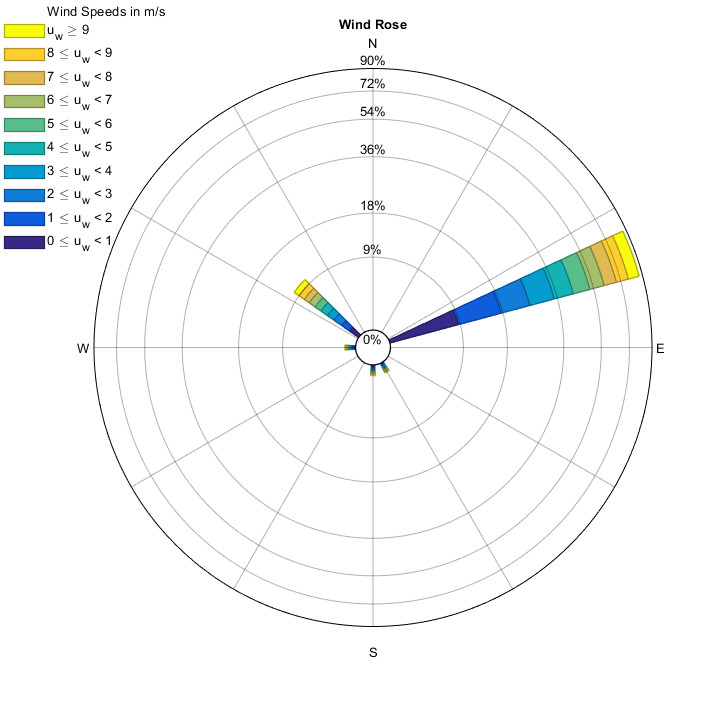[figure_handle, count, speeds, directions, Table, Others] = WindRose(DIR, SPD, 'logscale', true, 'logfactor', 1000);
% Notice that a very high logfactor adds more frequency circles. This can be prevented by specifying the number of frequencies to be shown (nFreq) or the frequency values to be shonw (freqs)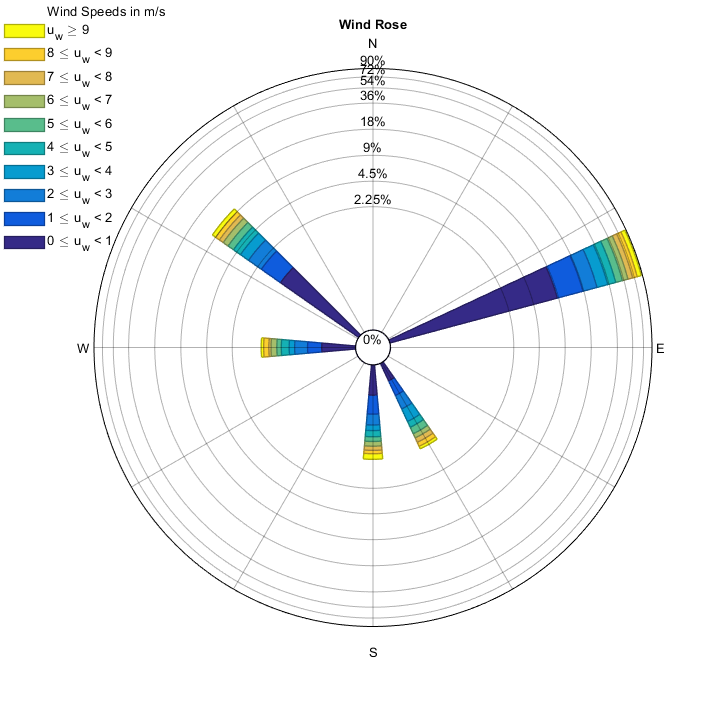## Subplot

### 'axes'

Create wind roses in different axes (in the same or in different figures).

To create wind roses in different axes use the 'axes' argument, followed by the handle of the axes in which to plot the wind roses. Different color maps can be used in each wind rose without extra code.

				rng(22081983);
[spd2, dir2] = WindRandomDistrib(8760, 20);
rng(02062020);
[spd3, dir3] = WindRandomDistrib(8760, 20);

Options = {'anglenorth', 0, 'angleeast', 90, 'labels', {'N (0°)', 'E (90°)', 'S (180°)', 'W (270°)'}, 'freqlabelangle', 45, 'legendtype', 0};


Option 1: Call the subplot and set the wind rose axes to be Current axes (gca) (this should be the favorite for almost everyone).

					subplot(2, 2, 1); set(gcf, 'units', 'normalized', 'position', [0 0 1 1]);
Options1 = [Options, {'axes', gca, 'cmap', 'jet'}]; % This is the simplest option to concatenate cell arrays. It's the same as using Options1 = {'legendtype',0,'axes',gca};
[figure_handle, count1, speeds1, directions1, Table1] = WindRose(dir, spd, Options1);


Option 2: Assign the subplot/subaxes handle to a variable.

					axes1 = subplot(2, 2, 2);
Options2 = [Options, {'axes', axes1, 'cmap', 'parula'}];
[figure_handle, count2, speeds2, directions2, Table2] = WindRose(dir2, spd2, Options2);


Option3: Call the subplot inside the options directly.

					Options3 = [Options, {'axes', subplot(2, 2, 3), 'cmap', 'invbone'}];
[figure_handle, count3, speeds3, directions3, Table3] = WindRose(dir3, spd3, Options3);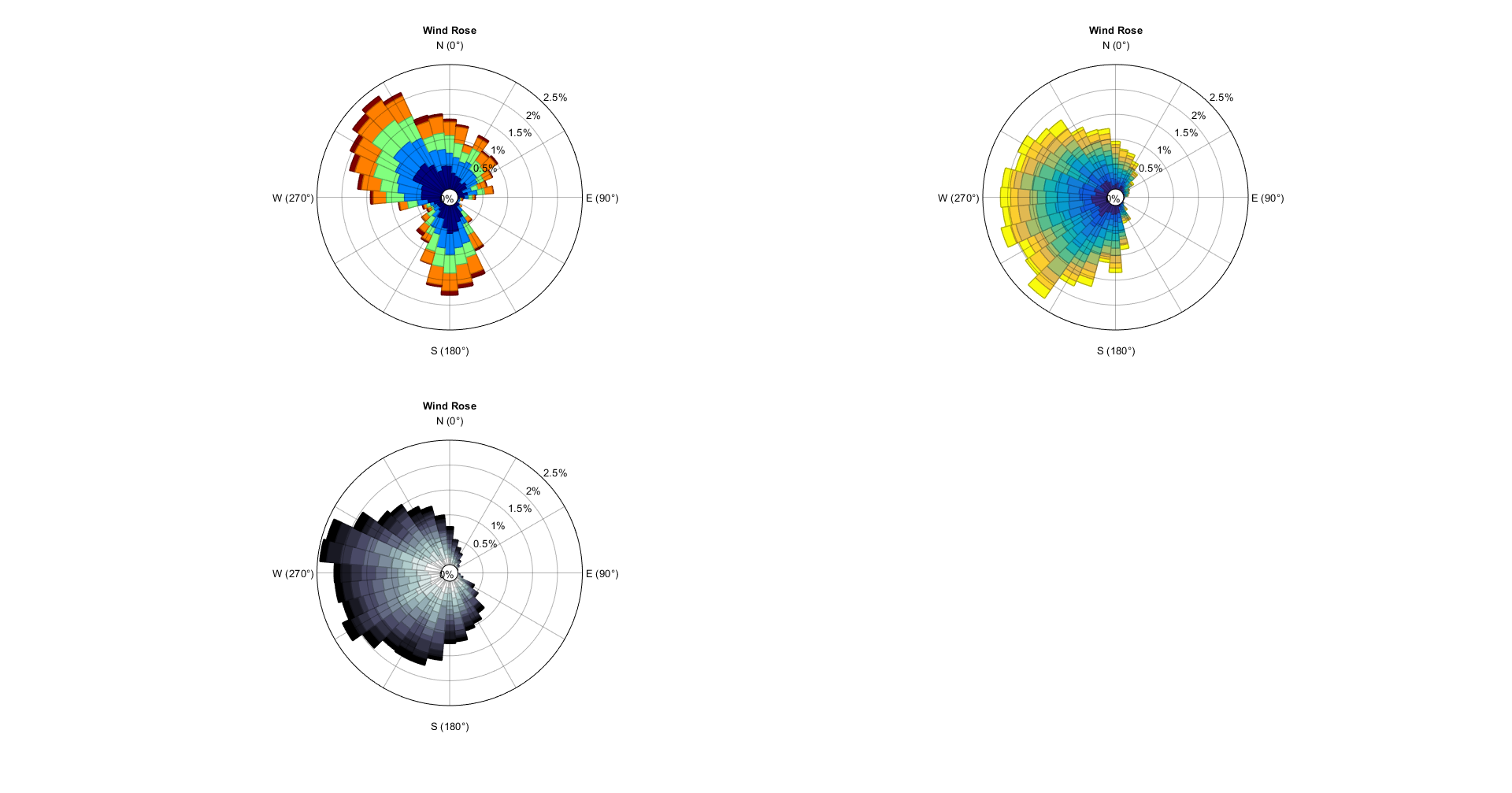## Tiledlayout

Use tiledlayout option to create a frame and plot windroses on it

In order to use tiledlayout, the easiest option is to draw an empty plot inside the tile and then call the WindRose with the axes option:

				tiledlayout(2,2);
nexttile; plot([],[]); WindRose(dir,spd,'axes',gca,'cmap','parula','legendtype',0);
nexttile; plot([],[]); WindRose(dir,spd,'axes',gca,'cmap','winter','legendtype',0);
nexttile; plot([],[]); WindRose(dir,spd,'axes',gca,'cmap','jet','legendtype',0);
nexttile; plot([],[]); WindRose(dir,spd,'axes',gca,'cmap','hsv','legendtype',0);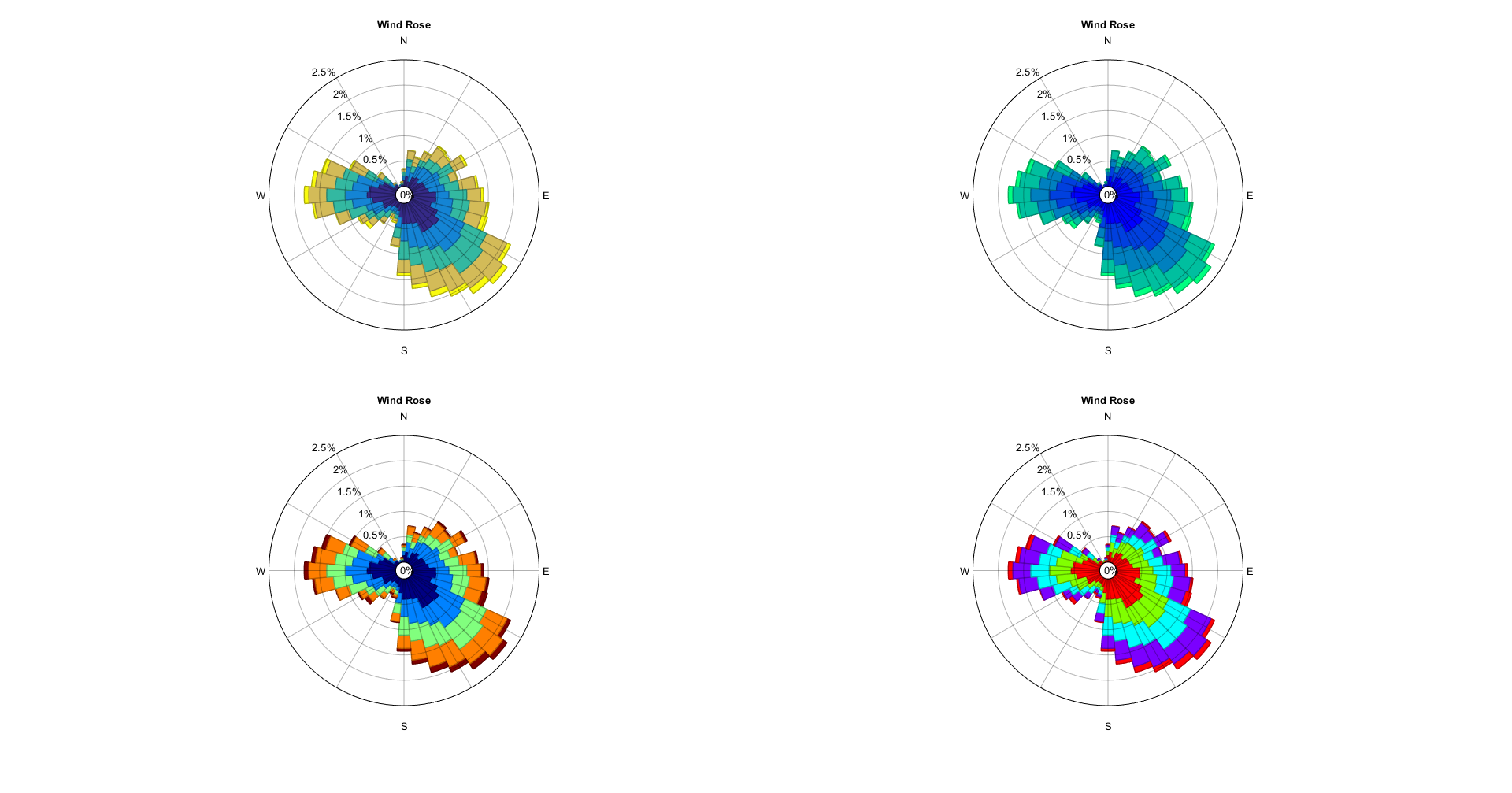## Text font face

### 'textfontname', 'titlefontname', 'legendfontname'

Change the font face for labels, title and legend.

It is also possible to change text fonts, separately for labels ('textfontname'), title ('titlefontname') and legend ('legendfontname'). The default font face for every text is Helvetica.

				Options4.textfontname = 'Arial Black';
Options4.titlefontname = 'Courier New';
Options4.legendfontname = 'Calibri Light';
Options4.cmap = 'spring';
[figure_handle, count3, speeds3, directions3, Table3] = WindRose(dir, spd, Options4);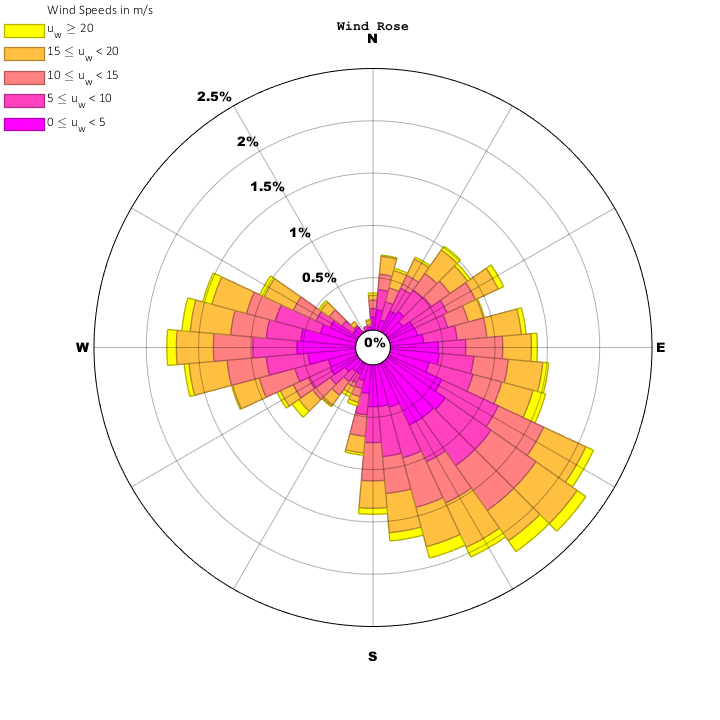## Title font weight

### 'TitleFontWeight'

Change the title font weight.

If you want the title to appear with a different weight (other than bold) you can choose between 'normal', 'light', 'demi' and 'bold':

				[figure_handle, count, speeds, directions, Table] = WindRose(dir, spd, 'TitleFontWeight', 'demi');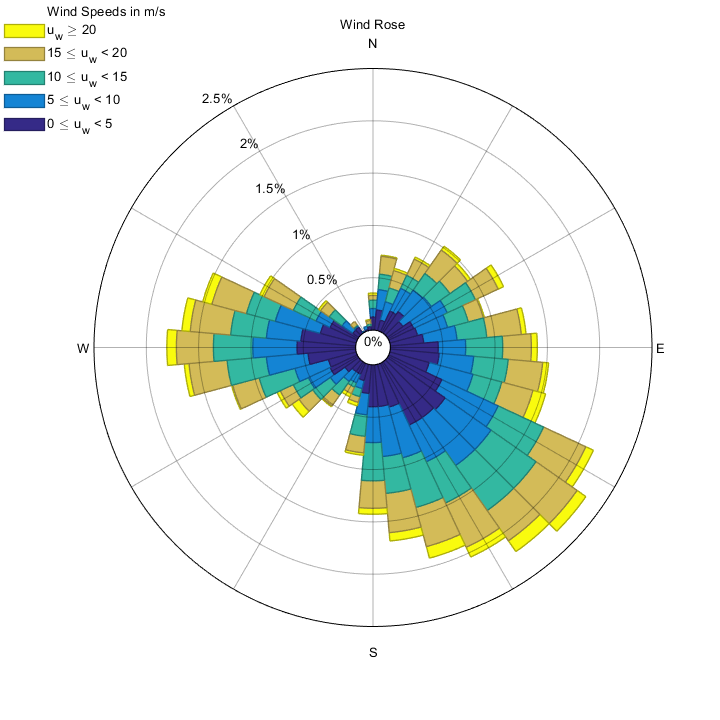## Text Appearance (Font, color, weight, angle, size)

Change the style of any of the text labels appearing on the figure (title, axes labels, frequency labels, legend and colorbar).

You can change the styles by changing the value of any of the following variables:

ParameterValue TypeDefault valueResult
'frequencyfontsize'Numeric.Matlab's defaultChanges Frequency Labels Font Size
'frequencyfontname'String.Matlab's defaultChanges Frequency Labels Font (use command listfonts to list available fonts)
'frequencyfontweight'String.'normal'Changes Frequency Labels Font Weight (normal/bold/light/demi)
'frequencyfontangle'String.'normal'Changes Frequency Labels Font Angle (normal/italic)
'frequencyfontcolor'Matlab color.'k'Changes Frequency Labels Font Color
'axesfontsize'Numeric.Matlab's defaultChanges Axes Labels (N,E,S,W) Font Size
'axesfontname'String.Matlab's defaultChanges Axes Labels (N,E,S,W) Font (use command listfonts to list available fonts)
'axesfontweight'String.'normal'Changes Axes Labels (N,E,S,W) Font Weight (normal/bold)
'axesfontangle'String.'normal'Changes Axes Labels (N,E,S,W) Font Angle (normal/italic)
'axesfontcolor'Matlab color.'k'Changes Axes Labels (N,E,S,W) Font Color
'titlefontsize'Numeric.Matlab's defaultChanges Figure Title Font Size
'titlefontname'String.Matlab's defaultChanges Figure Title Font (use command listfonts to list available fonts)
'titlefontweight'String.'normal'Changes Figure Title Font Weight (normal/bold)
'titlefontangle'String.'normal'Changes Figure Title Font Angle (normal/italic)
'titlecolor'Matlab color.'k'Changes Figure Title Font Color
'legendfontsize'Numeric.Matlab's defaultChanges Legend & Legend type 1 title Font Size
'legendfontname'String.Matlab's defaultChanges Legend & Legend type 1 title Font (use command listfonts to list available fonts)
'legendfontweight'String.'normal'Changes Legend & Legend type 1 title Font Weight (normal/bold)
'legendfontangle'String.'normal'Changes Legend & Legend type 1 title Font Angle (normal/italic)
'legendcolor'Matlab color.'k'Changes Legend & Legend type 1 title Font Color
'legendbarfontsize'Numeric.Matlab's defaultChanges Legend Type 1 Colorbar Font Size => If not set, the value used will be equal to legendfontsize
'legendbarfontname'String.Matlab's defaultChanges Legend Type 1 Colorbar Font (use command listfonts to list available fonts) => If not set, the value used will be equal to legendfontname
'legendbarfontweight'String.'normal'Changes Legend Type 1 Colorbar Font Weight (normal/bold) => If not set, the value used will be equal to legendfontweight
'legendbarfontangle'String.'normal'Changes Legend Type 1 Colorbar Font Angle (normal/italic) => If not set, the value used will be equal to legendfontangle
'legendbarcolor'Matlab color.'k'Changes Legend Type 1 Colorbar Color (numbers and box) => If not set, the value used will be equal to legendcolor

Note that using any of these parameters will override the values set with 'TextColor' or 'TextFontName'.

                Options.TextColor = 'k'; % This will be overridden
Options.TextFontName = 'Poor Richard'; % This will be overridden

Options.FrequencyFontColor = 'r';
Options.FrequencyFontWeight = 'bold';
Options.FrequencyFontName = 'Comic Sans MS';
Options.FrequencyFontSize = 12;
Options.FrequencyFontAngle = 'italic';

Options.AxesFontColor = 'b';
Options.AxesFontWeight = 'bold';
Options.AxesFontName = 'Rockwell Extra Bold';
Options.AxesFontSize = 15;
Options.AxesFontAngle = 'normal';

Options.TitleColor = [1 0.7 0.7];
Options.TitleFontSize = 20;
Options.TitleFontWeight = 'normal';
Options.TitleFontName = 'Jokerman';

Options.LegendColor = [1 0.4 0];
Options.LegendFontSize = 25;
Options.LegendFontWeight = 'normal';
Options.LegendFontName = 'Calibri';
Options.LegendFontAngle = 'italic’;

Options.LegendBarColor = [0 0.8 0];
Options.LegendBarFontSize = 9;
Options.LegendBarFontWeight = 'demi';
Options.LegendBarFontAngle = 'normal';
Options.LegendBarFontName = 'Gill Sans Ultra Bold Condensed';

Options.Labels = {'N','NE','E','SE','S','SW','W','NW'};
Options.legendtype = 1;

[figure_handle,count,speeds,directions,Table,Others] = WindRose(dir,spd,Options);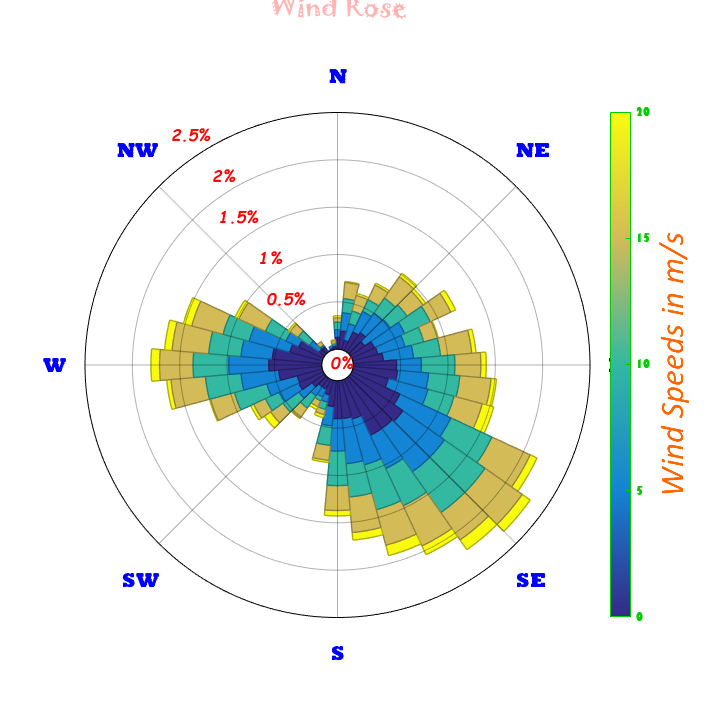## Gap between windrose arms

### 'gap'

Leave a gap between neighboring arms/sectors of the wind rose..

If you want the wind rose arms to be separated from one another, use the 'gap' parameter, setting the value in the range 0 (No separation) to 1 (All the space is for the gap, so no wind rose appears). Not how well this spacing combine with no edge in the patches:

				[figure_handle,count,speeds,directions,Table,Others] = WindRose(dir,spd,'gap',0.2,'edgecolor','none');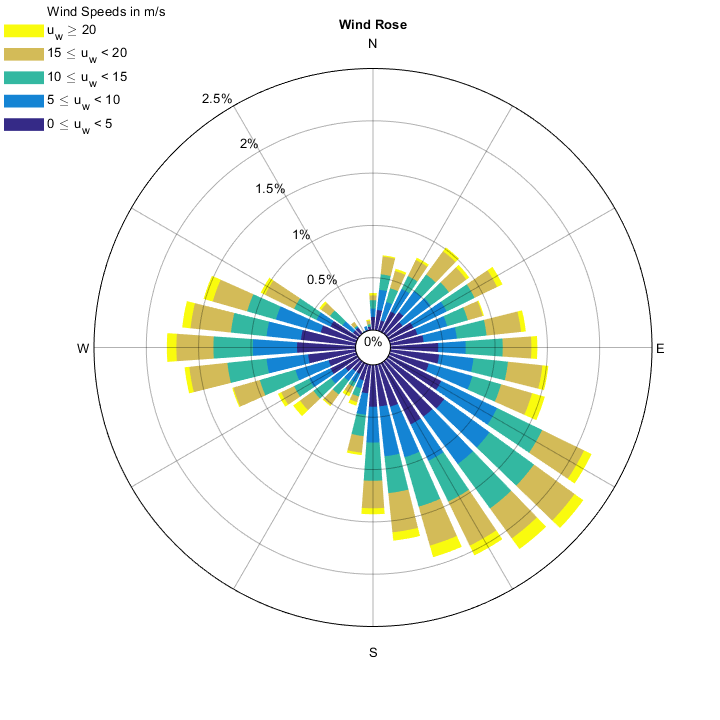A bigger value for the gap parameter will reduce the size of the Wind Rose’s arms:

				[figure_handle,count,speeds,directions,Table,Others] = WindRose(dir,spd,'gap',0.8);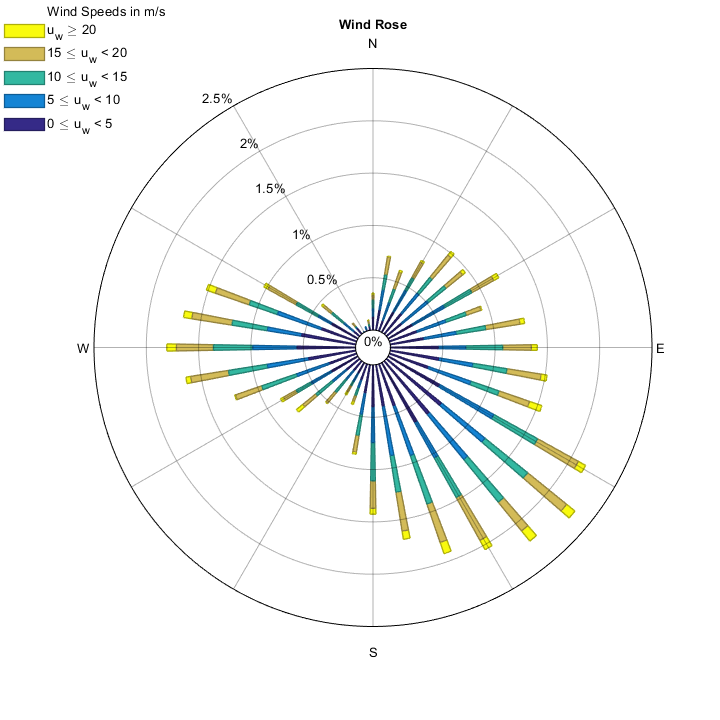See how a high value for the gap parameter (0.8) makes the arms much thinner and less visible.

Values are automatically clipped by the program between 0 and 1.

## Opacity/Transparency for patches and edges

### 'facealpha', 'edgealpha'

Set the opacity/transparency of the wind rose's patches (not the axes, whose opacity/transparency can be set independently with 'radialGridAlpha' and 'circularGridAlpha').

If you like the wind rose's patches/arms/sectors to be transparent, use the property 'faceAlpha' followed by a double in the range 0 (totally transparent) to 1 (totally opaque). Similary, the edges of the patches transparency/opacity can be set using 'edgeAlpha'.

				[figure_handle, count, speeds, directions, Table, Others] = WindRose(dir,spd,'faceAlpha',0.7,'edgeAlpha',0.3,'EdgeWidth',8,'gap',0.25,'Ndirections',6); % EdegWidth, gap and Ndirections specified, so the edgealpha is noticeable.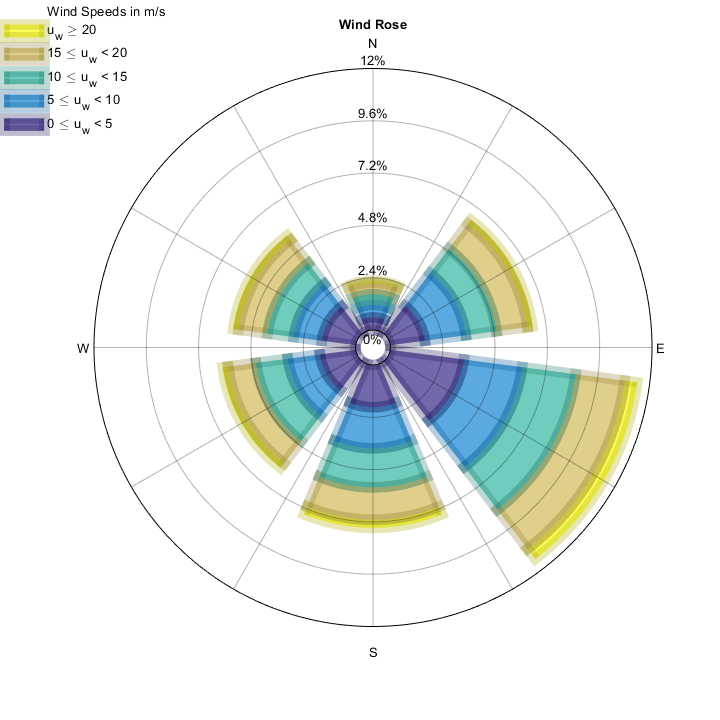## Plot wind roses in current axis given x,y coordinates

### 'X','Y'

Plot a wind rose at any place, given X & Y coordinates in current axis.

If you want to plot a wind rose at any position given the center coordinates 'X', 'Y', you might want to combine this with the 'scalefactor' parameter.

Wind Roses have a radius of 1, so if you set the 'scalefactor' to be 6, the new outer radius will be 6 (Wind Rose ranging from -6 to 6).

The following example plots three wind roses at positions (5,6), (-1,-2) and (4,1) respectively. The sizes (Scale factor) will be 1, 2 and 0.75 respectively. The example displays only 1 frequency value (the highest one) and removes the labels, but we want to preserve the 12 radial grids:

				figure;
Options5.freqlabelangle = 'auto';
Options5.nfreq = 1;
Options5.labels = '';

Options5.X = 5;
Options5.Y = 6;
[figure_handle, count, speeds, directions, Table] = WindRose(dir, spd, Options5);

Options5.X = -1;
Options5.Y = -2;
Options5.scalefactor = 2;
[figure_handle, count, speeds, directions, Table] = WindRose(dir, spd, Options5);

Options5.X = 4;
Options5.Y = 1;
Options5.scalefactor = 0.75;
[figure_handle, count, speeds, directions, Table] = WindRose(dir, spd, Options5);

grid on; box on; set(gca,'color',get(gcf,'color'));
ylim([-5 8]);
drawnow; set(gcf,'windowstate','maximized');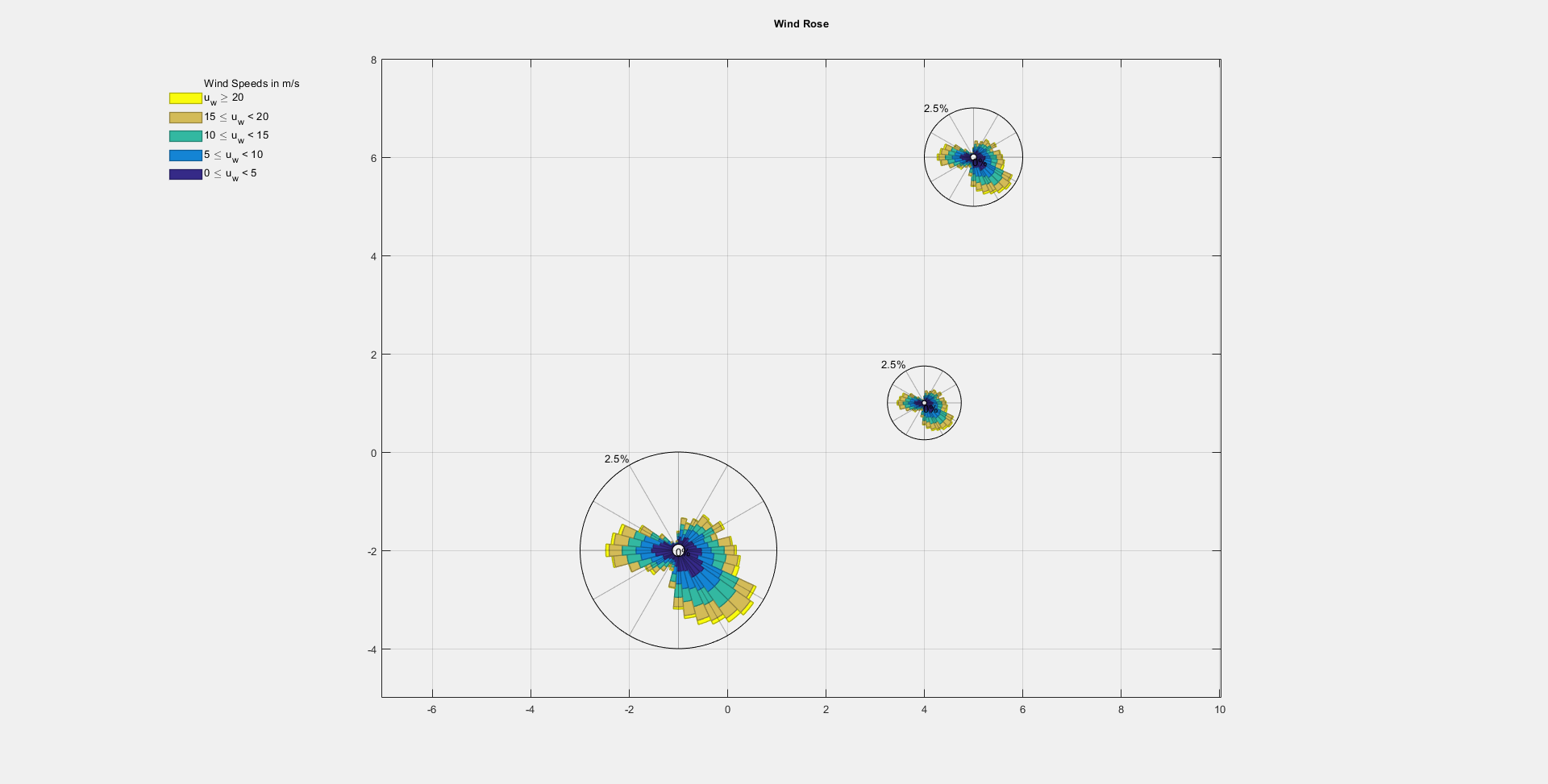See the three wind roses plotted at the specified positions and with the specified scale factor. Note that the wind rose with scale factor of 1 takes 2 units (2 radius with a length of 1 each), while the wind rose with scale factor of 2 takes 4 units (2 radius, with a length of 2 each).

## Plot extra stuff on a existing WindRose

Plot anything else over an existing wind rose.

Once we have plotted our wind rose, we might need plotting extra things over it. It is important to note that the wind rose ALWAYS present a radius of 1 unit multiplied by the scale factor (which is 1, by default). Then, whatever we need to plot, must consider this wind rose size.

It is not necessary to call 'hold on', because it is already called inside the wind rose.

				Options6.freqlabelangle = 'auto';
[figure_handle, count, speeds, directions, Table] = WindRose(dir, spd, Options6);

x = linspace(0,1,150)*2 - 1;
y = cos((x+1)*2*pi);
plot(x,y,'k','linewidth',3,'HandleVisibility','off'); % Do not display in the legend ('HandleVisivility','off')

quiver(0,0,0.6084,-0.4987,0,'filled','color','r','linewidth',3,'displayname','VECTOR!'); % Display a custom name in the legend ('displayname','my custom name')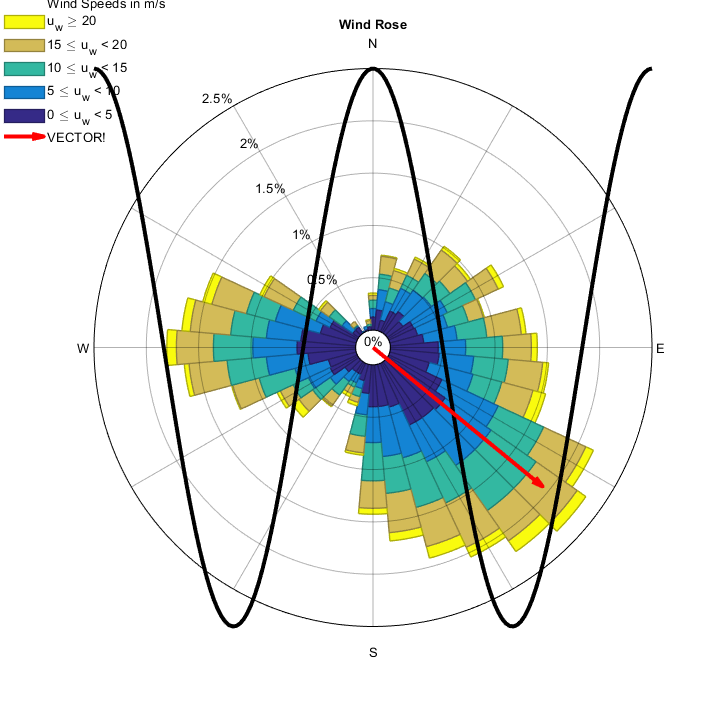## Outputs

This function has several outputs.

In order to specify dimensions of each array, the following shorthands have been used:

• s: Number of different speed bins shown in the wind rose
• d: Number of different direction bins shown in the wind rose
1. figure_handle: DOUBLE. Dimensions 1×1. The first one is the figure handle, which can be used to modify its position, printing, image saving, etc...
2. count: DOUBLE. Dimensions d×s. count is a two dimensional array, where the frequency for each intensity (in columns) and direction (in rows) is represented.
3. speeds: DOUBLE. Dimensions 1×s. speeds returns a 1-D array with the speeds appearing in the legends. The number of elements in this array matches the number of columns in count
4. directions: DOUBLE. Dimensions d×1. directions returns a 1-D array with the mean value of the directions of each "branch" in the wind rose. The number of elements in this array matches the number of rows in count
5. Table: CELL. Dimensions 4+d × 3+s. Table is a summary table where the frequencies for each direction and each speed are shown, in an excel ready format, so this can be used for xlswrite or just prompting in Matlab's command window.
6. Others: STRUCTURE. Dimensions 41×1. A structure with several parameters that can be useful to generate similar WindRoses. For the moment, this structure includes:

a)  Others.MaxFrequency: DOUBLE. Dimensions 1×1. Maximum frequency of the outer ring.

b)  Others.nFrequencies: DOUBLE. Dimensions 1×1. Number of frequency rings appearing in the figure.

c)  Others.freqs: DOUBLE. Dimensions 1×n. Value (in percentage) of frequency rings appearing in the figure.

## Analysis Mode

The function allows to calculate its output without actually showing the figure.

This can be helpful if you need obtaining the summary table or the maximum frequency and number of frequency rings that would be displayed in the figure with the current input arguments.

For example, this analysis mode can be used to iterate through different data series in order to obtain the maximum of all the frequencies, and the number of frequency grids in that case, so whenever plotting all the figures, the maximum frequency can be fixed at the same value for all the wind roses, therefore obtaining similar plots.

The analysis mode can be called as follows, combined with any other parameter and using the Options argument as well:

            [figure_handle,count,speeds,directions,Table,Others] = WindRose(direction,speed,'analysismode',true);


The figure_handle output will be an empty array (0×0) since no figure is created in the analysis mode.

## Author, Contact & Donations